# BGVAR: Bayesian Global Vector Autoregression

#### 07 April 2022

Abstract

This document describes the BGVAR library to estimate Bayesian Global vector autoregressions (GVAR) with different prior specifications and stochastic volatility. The library offers a fully fledged toolkit to conduct impulse response functions, forecast error variance and historical error variance decompositions. To identify structural shocks in a given country model or joint regional shocks, the library offers simple Cholesky decompositions, generalized impulse response functions and zero and sign restrictions – the latter of which can also be put on the cross-section. We also allow for different structures of the GVAR like including different weights for different variables or setting up additional country models that determine global variables such as oil prices. Last, we provide functions to conduct and evaluate out-of-sample forecasts as well as conditional forecasts that allow for the setting of a future path for a particular variable of interest. The toolbox requires R>=3.5.

# Introduction

This vignette describes the BGVAR package that allows for the estimation of Bayesian global vector autoregressions (GVARs). The focus of the vignette is to provide a range of examples that demonstrate the full functionality of the library. It is accompanied by a more technical description of the GVAR framework. Here, it suffices to briefly summarize the main idea of a GVAR, which is a large system of equations designed to analyze or control for interactions across units. Most often, these units refer to countries and the interactions between them arise through economic and financial interdependencies. Also in this document, the examples we provide contain cross-country data. In principle, however, the GVAR framework can be applied to other units, such as regions, firms, etc. The following examples show how the GVAR can be used to either estimate spillover effects from one country to another, or alternatively, to look at the effects of a domestic shock controlling for global factors.

In a nutshell, the GVAR consists of two stages. In the first, $$N$$ vector autoregressive (VAR) models are estimated, one per unit. Each equation in a unit model is augmented with foreign variables, that control for global factors and link the unit-specific models later. Typically, these foreign variables are constructed using exogenous, bilateral weights, stored in an $$N \times N$$ weight matrix. The classical framework of Pesaran, Schuermann, and Weiner (2004) and Dees et al. (2007) proposes estimating these country models in vector error correction form, while in this package we take a Bayesian stance and estimation is carried out using VARs. The user can transform the data prior estimation into stationary form or estimate the model in levels. The BGVAR package also allows us to include a trend to get trend-stationary data. In the second step, the single country models are combined using the assumption that single models are linked via the exogenous weights, to yield a global representation of the model. This representation of the model is then used to carry out impulse response analysis and forecasting.

This vignette consists of four blocks: getting started and data handling, estimation, structural analysis and forecasting. In the next part, we discuss which data formats the bgvar library can handle. We then proceed by showing examples of how to estimate a model using different Bayesian shrinkage priors – for references see Crespo Cuaresma, Feldkircher, and Huber (2016) and Feldkircher and Huber (2016). We also discuss how to run diagnostic and convergence checks and examine the main properties of the model. In the third section, we turn to structural analysis, either using recursive (Cholesky) identification or sign restrictions. We will also discuss structural and generalized forecast error variance decompositions and historical decompositions. In the last section, we show how to compute unconditional and conditional forecasts with the package.

# Getting Started

We start by installing the package from CRAN and attaching it with

oldpar <- par(no.readonly=TRUE)
set.seed(1)
library(BGVAR)
## Warning: package 'BGVAR' was built under R version 4.0.5

To ensure reproducibility of the examples that follow, we have set a particular seed (for Rs random number generator). As every R library, the BGVAR package provides built-in help files which can be accessed by typing ? followed by the function / command of interest. It also comes along with four example data sets, two of them correspond to data the quarterly data set used in Feldkircher and Huber (2016) (eerData, eerDataspf), one is on monthly frequency (monthlyData). For convenience we also include the data that come along with the Matlab GVAR toolbox of Smith, L.V. and Galesi, A. (2014), pesaranData. We include the 2019 vintage (Mohaddes and Raissi 2020).

We start illustrating the functionality of the BGVAR package by using the eerData data set from Feldkircher and Huber (2016). It contains 76 quarterly observations for 43 countries over the period from 1995Q1 to 2013Q4. The euro area (EA) is included as a regional aggregate.

We can load the data by typing

data(eerData)

This loads two objects: eerData, which is a list object of length $$N$$ (i.e., the number of countries) and W.trade0012, which is an $$N \times N$$ weight matrix.

We can have a look at the names of the countries contained in eerData

names(eerData)
##   "EA" "US" "UK" "JP" "CN" "CZ" "HU" "PL" "SI" "SK" "BG" "RO" "EE" "LT" "LV"
##  "HR" "AL" "RS" "RU" "UA" "BY" "GE" "AR" "BR" "CL" "MX" "PE" "KR" "PH" "SG"
##  "TH" "IN" "ID" "MY" "AU" "NZ" "TR" "CA" "CH" "NO" "SE" "DK" "IS"

and at the names of the variables contained in a particular country by

colnames(eerData$UK) ##  "y" "Dp" "rer" "stir" "ltir" "tb" We can zoom in into each country by accessing the respective slot of the data list: head(eerData$US)
##             y          Dp      rer   stir   ltir           tb     poil
## [1,] 4.260580 0.007173874 4.535927 0.0581 0.0748 -0.010907595 2.853950
## [2,] 4.262318 0.007341077 4.483116 0.0602 0.0662 -0.010637081 2.866527
## [3,] 4.271396 0.005394799 4.506013 0.0580 0.0632 -0.007689327 2.799958
## [4,] 4.278025 0.006218849 4.526343 0.0572 0.0589 -0.008163675 2.821479
## [5,] 4.287595 0.007719866 4.543933 0.0536 0.0591 -0.008277170 2.917315
## [6,] 4.301597 0.008467671 4.543933 0.0524 0.0672 -0.009359032 2.977115

Here, we see that the global variable, oil prices (poil) is attached to the US country model. This corresponds to the classical GVAR set-up used among others in Pesaran, Schuermann, and Weiner (2004) and Dees et al. (2007). We also see that in general, each country model $$i$$ can contain a different set of variables $$k_i$$ as opposed to requirements in a balanced panel.

The GVAR toolbox relies on one important naming convention, though: It is assumed that neither the country names nor the variable names contain a . [dot]. The reason is that the program internally has to collect and separate the data more than once and in doing that, it uses the . to separate countries / entities from variables. To give a concrete example, the slot in the eerData list referring to the USA should not be labelled U.S.A., nor should any of the variable names contain a .

The toolbox also allows the user to submit the data as a $$T \times k$$ data matrix, with $$k=\sum^N_{i=1} k_i$$ denoting the sum of endogenous variables in the system. We can switch from data representation in list form to matrix from by using the function list_to_matrix (and vice versa using matrix_to_list).

To convert the eerData we can type:

bigX<-list_to_matrix(eerData)

For users who want to submit data in matrix form, the above mentioned naming convention implies that the column names of the data matrix have to include the name of the country / entity and the variable name, separated by a . For example, for the converted eerData data set, the column names look like:

colnames(bigX)[1:10]
##   "EA.y"    "EA.Dp"   "EA.rer"  "EA.stir" "EA.ltir" "EA.tb"   "US.y"
##   "US.Dp"   "US.rer"  "US.stir"

with the first part of each columname indicating the country (e.g., EA) and the second the variable (e.g., y), separated by a . Regardless whether the data are submitted as list or as big matrix, the underlying data can be either of matrix class or time series classes such as ts or xts.

Finally, we look at the second important ingredient to build our GVAR model, the weight matrix. Here, we use annual bilateral trade flows (including services), averaged over the period from 2000 to 2012. This implies that the $$ij^{th}$$ element of $$W$$ contains trade flows from unit $$i$$ to unit $$j$$. These weights can also be made symmetric by calculating $$\frac{(W_{ij}+W_{ji})}{2}$$. Using trade weights to establish the links in the GVAR goes back to the early GVAR literature (Pesaran, Schuermann, and Weiner 2004) but is still used in the bulk of GVAR studies. Other weights, such as financial flows, have been proposed in Eickmeier and Ng (2015) and examined in Feldkircher and Huber (2016). Another approach is to use estimated weights as in Feldkircher and Siklos (2019). The weight matrix should have rownames and colnames that correspond to the $$N$$ country names contained in Data.

head(W.trade0012)
##           EA         US         UK         JP         CN          CZ
## EA 0.0000000 0.13815804 0.16278169 0.03984424 0.09084817 0.037423312
## US 0.1666726 0.00000000 0.04093296 0.08397042 0.14211997 0.001438531
## UK 0.5347287 0.11965816 0.00000000 0.02628600 0.04940218 0.008349458
## JP 0.1218515 0.21683444 0.02288576 0.00000000 0.22708532 0.001999762
## CN 0.1747925 0.19596384 0.02497009 0.15965721 0.00000000 0.003323641
## CZ 0.5839067 0.02012227 0.03978617 0.01174212 0.03192080 0.000000000
##             HU          PL           SI           SK           BG           RO
## EA 0.026315925 0.046355019 0.0088805499 0.0140525286 0.0054915888 0.0147268739
## US 0.001683935 0.001895003 0.0003061785 0.0005622383 0.0002748710 0.0007034870
## UK 0.006157917 0.012682611 0.0009454295 0.0026078946 0.0008369228 0.0031639564
## JP 0.002364775 0.001761420 0.0001650431 0.0004893263 0.0001181310 0.0004293428
## CN 0.003763771 0.004878752 0.0005769658 0.0015252866 0.0006429077 0.0019212312
## CZ 0.025980933 0.062535144 0.0058429207 0.0782762640 0.0027079942 0.0080760690
##              EE           LT           LV           HR           AL
## EA 0.0027974288 3.361644e-03 1.857555e-03 0.0044360005 9.328127e-04
## US 0.0002678272 4.630261e-04 2.407372e-04 0.0002257508 2.057213e-05
## UK 0.0009922865 1.267497e-03 1.423142e-03 0.0004528439 3.931010e-05
## JP 0.0002038361 9.363053e-05 9.067431e-05 0.0001131534 4.025852e-06
## CN 0.0004410996 5.033345e-04 4.041432e-04 0.0006822057 1.435258e-04
## CZ 0.0009807047 2.196688e-03 1.107030e-03 0.0027393932 1.403948e-04
##              RS         RU           UA           BY           GE          AR
## EA 2.430815e-03 0.06112681 0.0064099317 1.664224e-03 0.0003655903 0.005088057
## US 7.079076e-05 0.01024815 0.0008939856 2.182909e-04 0.0001843835 0.004216105
## UK 2.730431e-04 0.01457675 0.0010429571 4.974630e-04 0.0001429294 0.001387324
## JP 2.951168e-05 0.01725841 0.0007768546 4.392221e-05 0.0001062724 0.001450359
## CN 1.701277e-04 0.02963668 0.0038451574 5.069157e-04 0.0001685347 0.005817928
## CZ 1.638111e-03 0.03839817 0.0082099438 1.447486e-03 0.0002651330 0.000482138
##             BR           CL          MX           PE          KR           PH
## EA 0.018938315 0.0051900915 0.011455138 0.0019463003 0.018602006 0.0034965601
## US 0.020711342 0.0064996880 0.140665729 0.0037375799 0.033586979 0.0076270807
## UK 0.007030657 0.0016970182 0.003832710 0.0005204850 0.010183755 0.0025279396
## JP 0.010910951 0.0077091104 0.011164908 0.0020779123 0.079512267 0.0190560664
## CN 0.025122526 0.0102797021 0.010960261 0.0040641411 0.103135774 0.0150847984
## CZ 0.001898955 0.0002425703 0.001538938 0.0001152155 0.005248484 0.0008790869
##             SG          TH          IN           ID          MY          AU
## EA 0.012319690 0.007743377 0.016629452 0.0065409485 0.009631702 0.010187442
## US 0.017449474 0.012410910 0.014898397 0.0079866535 0.017364286 0.011578348
## UK 0.011309096 0.006146707 0.013461838 0.0032341466 0.006768142 0.012811822
## JP 0.029885052 0.044438961 0.010319951 0.0369586674 0.035612054 0.047921306
## CN 0.029471018 0.024150334 0.024708981 0.0201353186 0.033336788 0.036066785
## CZ 0.002867306 0.003136170 0.003568422 0.0009949029 0.002695855 0.001291178
##              NZ          TR          CA          CH          NO          SE
## EA 0.0017250647 0.028935117 0.012886035 0.065444998 0.025593188 0.041186900
## US 0.0023769166 0.004969085 0.213004968 0.013343786 0.003975441 0.006793041
## UK 0.0022119334 0.013099627 0.020699895 0.026581778 0.033700469 0.022629934
## JP 0.0048098149 0.002560745 0.020149431 0.010014273 0.002895884 0.004375537
## CN 0.0030625686 0.006578520 0.019121787 0.007657646 0.002804712 0.005853722
## CZ 0.0001703354 0.006490032 0.001808704 0.013363416 0.003843421 0.013673889
##             DK           IS
## EA 0.025035373 1.163498e-03
## US 0.003135419 2.750740e-04
## UK 0.013518119 1.119179e-03
## JP 0.003207880 2.637944e-04
## CN 0.003990731 7.806304e-05
## CZ 0.007521567 1.490167e-04

The countries in the weight matrix should be in the same order as in the data list:

all(colnames(W.trade0012)==names(eerData))
##  TRUE

The weight matrix should be row-standardized and the diagonal elements should be zero:

rowSums(W.trade0012)
## EA US UK JP CN CZ HU PL SI SK BG RO EE LT LV HR AL RS RU UA BY GE AR BR CL MX
##  1  1  1  1  1  1  1  1  1  1  1  1  1  1  1  1  1  1  1  1  1  1  1  1  1  1
## PE KR PH SG TH IN ID MY AU NZ TR CA CH NO SE DK IS
##  1  1  1  1  1  1  1  1  1  1  1  1  1  1  1  1  1
diag(W.trade0012)
## EA US UK JP CN CZ HU PL SI SK BG RO EE LT LV HR AL RS RU UA BY GE AR BR CL MX
##  0  0  0  0  0  0  0  0  0  0  0  0  0  0  0  0  0  0  0  0  0  0  0  0  0  0
## PE KR PH SG TH IN ID MY AU NZ TR CA CH NO SE DK IS
##  0  0  0  0  0  0  0  0  0  0  0  0  0  0  0  0  0

Note that through row-standardizing, the final matrix is typically not symmetric (even when using the symmetric weights as raw input).

In what follows, we restrict the dataset to contain only three countries, EA, US and RU and adjust the weight matrix accordingly. We do this only for illustrational purposes to save time and storage in this document:

cN<-c("EA","US","RU")
eerData<-eerData[cN]
W.list<-lapply(W.list,function(l){l<-apply(l[cN,cN],2,function(x)x/rowSums(l[cN,cN]))})

This results in the same dataset as available in testdata.

In order to make BGVAR easier to handle for users working and organising data in spreadsheets via Excel, we provide a own reader function relying on the readxl package. In this section we intend to provide some code to write the provided datasets to Excel spreadsheets, and to show then how to read the data from Excel. Hence, we provide an easy-to-follow approach with an example how the data should be organised in Excel.

We start by exporting the data to excel. The spreadsheet should be organised as follows. Each sheet consists of the data set for one particular country, hence the naming of the sheets with the country names is essential. In each sheet, you should provide the time in the first column of the matrix, followed by one column per variable. In the following, we will export the eerData data set to Excel:

time <- as.character(seq.Date(as.Date("1995-01-01"),as.Date("2013-10-01"),by="quarter"))

for(cc in 1:length(eerData)){
x <- coredata(eerData[[cc]])
rownames(x) <- time
write.xlsx(x = x, file="./excel_eerData.xlsx", sheetName = names(eerData)[cc],
col.names=TRUE, row.names=TRUE, append=TRUE)
}

which will create in your current working directory an excel sheet named excel_eerData.xlsx. This can then be read to R with the BGVAR package as follows:

eerData_read <- excel_to_list(file = "./excel_eerData.xlsx", first_column_as_time=TRUE, skipsheet=NULL, ...)

which creates a list in the style of the original eerData data set. The first argument file has to be valid path to an excel file. The second argument first_column_as_time is a logical indicating whether you provide as first column in each spreadsheet a time index, while the skipsheet argument can be specified to leave out specific sheets (either as vector of strings or numeric indices). If you want to transform the list object to a matrix, you can use the command list_to_matrix or to transform it back to a list with matrix_to_list:

eerData_matrix <- list_to_matrix(eerData_read)
eerData_list <- matrix_to_list(eerData_matrix)

# Estimation

The main function of the BGVAR package is its bgvar function. The unique feature of this toolbox is that we use Bayesian shrinkage priors with optionally stochastic volatility to estimate the country models in the GVAR. In its current version, three priors for the country VARs are implemented:

• Non-conjugate Minnesota prior (MN, Litterman 1986; Koop and Korobilis 2010)
• Stochastic Search Variable Selection prior (SSVS, George, Sun, and Ni 2008)
• Normal-Gamma prior (NG, Huber and Feldkircher 2019)

The first two priors are described in more detail in Crespo Cuaresma, Feldkircher, and Huber (2016). For a more technical description of the Normal-Gamma prior see Huber and Feldkircher (2019) and for an application in the GVAR context Feldkircher and Siklos (2019). For the variances we can assume homoskedasticity or time variation (stochastic volatility). For the latter, the library relies on the stochvol package of Kastner (2016).

We start with estimating our toy model using the NG prior, the reduced eerData data set and the adjusted W.trade0012 weight matrix:

 model.1<-bgvar(Data=eerData,
draws=100,
burnin=100,
plag=1,
prior="NG",
hyperpara=NULL,
SV=TRUE,
thin=1,
trend=TRUE,
hold.out=0,
eigen=1
)

The default prior specification in bgvar is to use the NG prior with stochastic volatility and one lag for both the endogenous and weakly exogenous variables (plag=1). In general, due to its high cross-sectional dimension, the GVAR can allow for very complex univariate dynamics and it might thus not be necessary to increase the lag length considerably as in a standard VAR (Burriel and Galesi 2018). The setting hyperpara=NULL implies that we use the standard hyperparameter specification for the NG prior; see the helpfiles for more details.

Other standard specifications that should be submitted by the user comprise the number of posterior draws (draws) and burn-ins (burnin, i.e., the draws that are discarded). To ensure that the MCMC estimation has converged, a high-number of burn-ins is recommended (say 15,000 to 30,000). Saving the full set of posterior draws can eat up a lot of storage. To reduce this, we can use a thinning interval which stores only a thin$$^{th}$$ draw of the global posterior output. For example, with thin=10 and draws=5000 posterior draws, the amount of MCMC draws stored is 500. TREND=TRUE implies that the model is estimated using a trend. Note that regardless of the trend specification, each equation always automatically includes an intercept term.

Expert users might want to take further adjustments. These have to be provided via a list (expert). For example, to speed up computation, it is possible to invoke parallel computing in R. The number of available cpu cores can be specified via cores. Ideally this number is equal to the number of units $$N$$ (expert=list(cores=N)). Based on the user’s operating system, the package then either uses parLapply (Windows platform) or mclapply (non-Windows platform) to invoke parallel computing. If cores=NULL, the unit models are estimated subsequently in a loop (via R’s lapply function). To use other / own apply functions, pass them on via the argument applyfun. As another example, we might be interested in inspecting the output of the $$N$$ country models in more detail. To do so, we could provide expert=list(save.country.store=TRUE), which allows to save the whole posterior distribution of each unit / country model. Due to storage reasons, the default is set to FALSE and only the posterior medians of the single country models are reported. Note that even in this case, the whole posterior distribution of the global model is stored.

We estimated the above model with stochastic volatility (SV=TRUE). There are several reasons why one may want to let the residual variances change over time. First and foremost, most time periods used in macroeconometrics are nowadays rather volatile including severe recessions. Hence accounting for time variation might improve the fit of the model (Primiceri 2005; Sims and Zha 2006; Dovern, Feldkircher, and Huber 2016; Huber 2016). Second, the specification implemented in the toolbox nests the homoskedastic case. It is thus a good choice to start with the more general case when first confronting the model with the data. For structural analysis such as the calculation of impulse responses, we take the variance covariance matrix with the median volatilities (over the sample period) on its diagonal. If we want to look at the volatilities of the first equation (y) in the euro area country model, we can type:

model.1$cc.results$sig$EA[,"EA.y","EA.y"] To discard explosive draws, we can compute the eigenvalues of the reduced form of the global model, written in its companion form. Unfortunately, this can only be done once the single models have been estimated and stacked together (and hence not directly built into the MCMC algorithm for the country models). To discard draws that lead to higher eigenvalues than 1.05, set eigen=1.05. We can look at the 10 largest eigenvalues by typing: model.1$stacked.results$F.eigen[1:10] ##  0.9835544 0.9523397 0.9795195 0.9907462 0.9863376 0.9955975 0.9789576 ##  0.9892011 0.9966557 0.9918312 Last, we have used the default option h=0, which implies that we use the full sample period to estimate the GVAR. For the purpose of forecast evaluation, h could be specified to a positive number, which then would imply that the last h observations are reserved as a hold-out sample and not used to estimate the model. ## Model Output and Diagnostic Checks Having estimated the model, we can summarize the outcome in various ways. First, we can use the print method print(model.1) ## --------------------------------------------------------------------------- ## Model Info: ## Prior: Normal-Gamma prior (NG) ## Number of lags for endogenous variables: 1 ## Number of lags for weakly exogenous variables: 1 ## Number of posterior draws: 100/1=100 ## Size of GVAR object: 0.5 Mb ## Trimming leads to 34 (34%) stable draws out of 100 total draws. ## --------------------------------------------------------------------------- ## Model specification: ## ## EA: y, Dp, rer, stir, ltir, tb, y*, Dp*, rer*, stir*, ltir*, tb*, poil**, trend ## US: y, Dp, rer, stir, ltir, tb, poil, y*, Dp*, rer*, stir*, ltir*, tb*, trend ## RU: y, Dp, rer, stir, tb, y*, Dp*, rer*, stir*, ltir*, tb*, poil**, trend This just prints the submitted arguments of the bgvar object along with the model specification for each unit. The asterisks indicate weakly exogenous variables, double asterisks exogenous variables and variables without asterisks the endogenous variables per unit. The summary method is a more enhanced way to analyze output. It computes descriptive statistics like convergence properties of the MCMC chain, serial autocorrelation in the errors and the average pairwise autocorrelation of cross-unit residuals.  summary(model.1) ## --------------------------------------------------------------------------- ## Model Info: ## Prior: Normal-Gamma prior (NG) ## Number of lags for endogenous variables: 1 ## Number of lags for weakly exogenous variables: 1 ## Number of posterior draws: 100/1=100 ## Number of stable posterior draws: 34 ## Number of cross-sectional units: 3 ## --------------------------------------------------------------------------- ## Convergence diagnostics ## Geweke statistic: ## 92 out of 360 variables' z-values exceed the 1.96 threshold (25.56%). ## --------------------------------------------------------------------------- ## F-test, first order serial autocorrelation of cross-unit residuals ## Summary statistics: ## ========= ========== ====== ## \ # p-values in % ## ========= ========== ====== ## >0.1 5 27.78% ## 0.05-0.1 1 5.56% ## 0.01-0.05 3 16.67% ## <0.01 9 50% ## ========= ========== ====== ## --------------------------------------------------------------------------- ## Average pairwise cross-unit correlation of unit-model residuals ## Summary statistics: ## ======= ======== ======== ========== ========== ======== ======== ## \ y Dp rer stir ltir tb ## ======= ======== ======== ========== ========== ======== ======== ## <0.1 0 (0%) 3 (100%) 2 (66.67%) 1 (33.33%) 2 (100%) 3 (100%) ## 0.1-0.2 0 (0%) 0 (0%) 0 (0%) 0 (0%) 0 (0%) 0 (0%) ## 0.2-0.5 3 (100%) 0 (0%) 1 (33.33%) 2 (66.67%) 0 (0%) 0 (0%) ## >0.5 0 (0%) 0 (0%) 0 (0%) 0 (0%) 0 (0%) 0 (0%) ## ======= ======== ======== ========== ========== ======== ======== ## --------------------------------------------------------------------------- We can now have a closer look at the output provided by summary. The header contains some basic information about the prior used to estimate the model, how many lags, posterior draws and countries. The next line shows Geweke’s CD statistic, which is calculated using the coda package. Geweke’s CD assesses practical convergence of the MCMC algorithm. In a nutshell, the diagnostic is based on a test for equality of the means of the first and last part of a Markov chain (by default we use the first 10% and the last 50%). If the samples are drawn from the stationary distribution of the chain, the two means are equal and Geweke’s statistic has an asymptotically standard normal distribution. The test statistic is a standard Z-score: the difference between the two sample means divided by its estimated standard error. The standard error is estimated from the spectral density at zero and so takes into account any autocorrelation. The test statistic shows that only a small fraction of all coefficients did not convergence. Increasing the number of burn-ins can help decreasing this fraction further. The statistic can also be calculated by typing conv.diag(model.1). The next model statistic is the likelihood of the global model. This statistic can be used for model comparison. Next and to assess, whether there is first order serial autocorrelation present, we provide the results of a simple F-test. The table shows the share of p-values that fall into different significance categories. Since the null hypothesis is that of no serial correlation, we would like to have as many large ($$>0.1$$) p-values as possible. The statistics show that already with one lag, serial correlation is modest in most equations’ residuals. This could be the case since we have estimated the unit models with stochastic volatility. To further decrease serial correlation in the errors, one could increase the number of lags via plag. The last part of the summary output contains a statistic of cross-unit correlation of (posterior median) residuals. One assumption of the GVAR framework is that of negligible, cross-unit correlation of the residuals. Significant correlations prohibit structural and spillover analysis (Dees et al. 2007). In this example, correlation is reasonably small. Some other useful methods the BGVAR toolbox offers contain the coef (or coefficients as its alias) methods to extract the $$k \times k \times plag$$ matrix of reduced form coefficients of the global model. Via the vcov command, we can access the global variance covariance matrix and the logLik() function allows us to gather the global log likelihood (as provided by the summary command). Fmat <- coef(model.1) Smat <- vcov(model.1) lik <- logLik(model.1) Last, we can have a quick look at the in-sample fit using either the posterior median of the country models’ residuals (global=FALSE) or those of the global solution of the GVAR (global=TRUE). The in-sample fit can also be extracted by using fitted(). Here, we show the in-sample fit of the euro area model (global=FALSE). yfit <- fitted(model.1) plot(model.1, global=FALSE, resp="EA")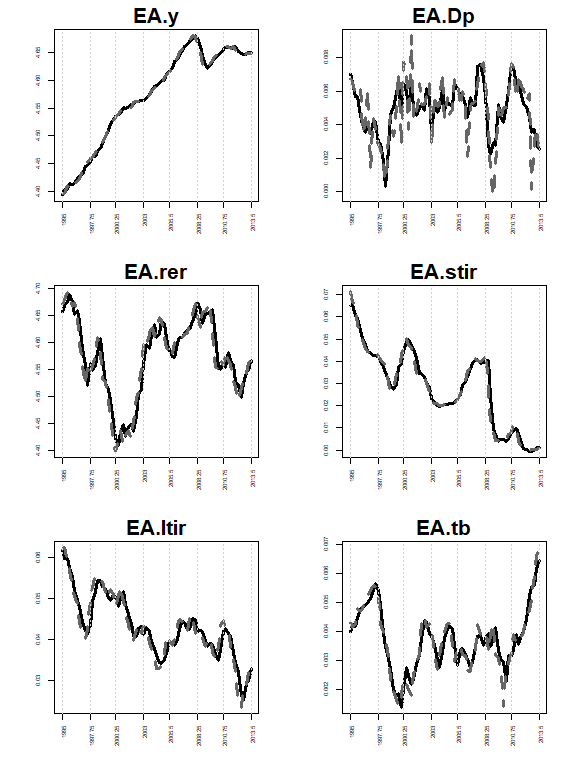In-sample fit for euro area variables We can estimate the model with two further priors on the unit models, the SSVS prior and the Minnesota prior. To give a concrete example, the SSVS prior can be invoked by typing:  model.ssvs.1<-bgvar(Data=eerData, W=W.trade0012, draws=100, burnin=100, plag=1, prior="SSVS", hyperpara=NULL, SV=TRUE, thin=1, trend=TRUE, eigen=1, expert=list(save.shrink.store=TRUE) ) One feature of the SSVS prior is that it allows us to look at the posterior inclusion probabilities to gauge the importance of particular variables. For example, we can have a look at the PIPs of the euro area model by typing: model.ssvs.1$cc.results$PIP$PIP.cc$EA ## y Dp rer stir ltir tb ## y_lag1 1.00 0.80 0.92 0.23 0.17 0.76 ## Dp_lag1 0.28 0.34 0.21 0.22 0.42 0.30 ## rer_lag1 0.53 0.41 1.00 1.00 0.23 0.68 ## stir_lag1 0.98 0.25 0.61 1.00 0.19 0.70 ## ltir_lag1 0.62 0.87 0.34 0.31 1.00 0.76 ## tb_lag1 0.28 0.33 0.37 0.31 0.18 1.00 ## y* 0.95 0.61 0.39 0.14 0.13 0.04 ## Dp* 0.25 0.26 0.20 0.03 0.09 0.54 ## rer* 0.10 0.22 1.00 0.65 0.16 0.27 ## stir* 0.18 0.85 0.14 0.08 0.22 0.16 ## ltir* 0.06 0.39 0.59 0.88 0.07 0.30 ## tb* 0.15 1.00 0.13 0.14 0.23 0.39 ## poil** 0.13 1.00 0.29 0.20 0.16 0.04 ## y*_lag1 0.43 0.25 0.54 0.19 0.11 0.69 ## Dp*_lag1 0.92 0.26 0.28 0.38 0.21 0.13 ## rer*_lag1 0.18 0.27 0.93 0.12 0.10 0.14 ## stir*_lag1 0.27 0.20 0.32 0.57 0.16 0.45 ## ltir*_lag1 1.00 1.00 0.47 1.00 0.29 0.50 ## tb*_lag1 0.09 1.00 0.65 0.88 0.23 0.68 ## poil**_lag1 0.15 0.73 0.48 0.23 0.08 0.13 ## cons 0.28 0.43 0.74 0.17 0.09 1.00 ## trend 0.89 0.65 0.56 0.04 0.23 1.00 The equations in the EA country model can be read column-wise with the rows representing the associated explanatory variables. The example shows that besides other variables, the trade balance (tb) is an important determinant of the real exchange rate (rer). We can also have a look at the average of the PIPs across all units: model.ssvs.1$cc.results$PIP$PIP.avg
##                      y        Dp       rer       stir  ltir        tb poil
## y_lag1      1.00000000 0.5266667 0.4000000 0.30333333 0.200 0.6200000 0.17
## Dp_lag1     0.20666667 0.5033333 0.1533333 0.18666667 0.285 0.3366667 0.34
## rer_lag1    0.24333333 0.5700000 1.0000000 0.70333333 0.185 0.8533333 0.98
## stir_lag1   0.99333333 0.1533333 0.4400000 1.00000000 0.140 0.3033333 0.29
## ltir_lag1   0.44000000 0.4650000 0.3050000 0.23500000 1.000 0.5000000 0.28
## tb_lag1     0.47333333 0.4833333 0.4866667 0.36000000 0.120 1.0000000 0.02
## y*          0.44666667 0.2500000 0.4866667 0.41333333 0.175 0.3733333 1.00
## Dp*         0.25333333 0.4766667 0.2366667 0.04666667 0.395 0.6333333 1.00
## rer*        0.46333333 0.4666667 0.7800000 0.40333333 0.580 0.4366667 0.34
## stir*       0.26333333 0.3933333 0.2300000 0.13666667 0.145 0.2366667 0.26
## ltir*       0.07333333 0.4766667 0.2866667 0.39333333 0.160 0.2166667 0.14
## tb*         0.32666667 0.8500000 0.1266667 0.16666667 0.200 0.3500000 1.00
## poil**      0.56500000 0.5600000 0.2950000 0.29000000 0.160 0.3850000  NaN
## y*_lag1     0.32000000 0.1300000 0.5466667 0.42000000 0.150 0.4000000 0.90
## Dp*_lag1    0.48000000 0.1566667 0.1566667 0.23333333 0.255 0.1600000 0.22
## rer*_lag1   0.34666667 0.4600000 0.8133333 0.10333333 0.550 0.2233333 0.10
## stir*_lag1  0.44666667 0.1733333 0.3333333 0.30333333 0.160 0.2900000 0.07
## ltir*_lag1  0.40666667 0.4066667 0.2533333 0.39000000 0.340 0.2866667 0.21
## tb*_lag1    0.15333333 0.7300000 0.2633333 0.40000000 0.215 0.3400000 0.23
## poil**_lag1 0.22500000 0.4100000 0.3750000 0.22000000 0.080 0.1400000  NaN
## cons        0.47000000 0.3100000 0.6100000 0.26000000 0.080 0.7066667 0.42
## trend       0.59666667 0.4033333 0.2333333 0.28000000 0.305 0.4733333 0.87
## poil_lag1   0.17000000 0.1400000 0.0700000 0.95000000 0.420 0.3000000 1.00

This shows that the same determinants for the real exchange rate appear as important regressors in other country models.

## Different Specifications of the Model

In this section we explore different specifications of the structure of the GVAR model. Other specification choices that relate more to the time series properties of the data, such as specifying different lags and priors are left for the reader to explore. We will use the SSVS prior and judge the different specifications by examining the posterior inclusion probabilities.

As a first modification, we could use different weights for different variable classes as proposed in Eickmeier and Ng (2015). For example we could use financial weights to construct weakly exogenous variables of financial factors and trade weights for real variables.

The eerData set provides us with a list of different weight matrices that are described in the help files.

Now we specify the sets of variables to be weighted:

variable.list<-list();variable.list$real<-c("y","Dp","tb");variable.list$fin<-c("stir","ltir","rer")

We can then re-estimate the model and hand over the variable.list via the argument expert:

# weights for first variable set tradeW.0012, for second finW0711
model.ssvs.2<-bgvar(Data=eerData,
plag=1,
draws=100,
burnin=100,
prior="SSVS",
SV=TRUE,
eigen=1,
expert=list(variable.list=variable.list,save.shrink.store=TRUE),
trend=TRUE
)

Another specification would be to include a foreign variable only when its domestic counterpart is missing. For example, when working with nominal bilateral exchange rates we probably do not want to include also its weighted average (which corresponds to something like an effective exchange rate). Using the previous model we could place an exclusion restriction on foreign long-term interest rates using Wex.restr which is again handed over via expert. The following includes foreign long-term rates only in those country models where no domestic long-term rates are available:

# does include ltir* only when ltir is missing domestically
model.ssvs.3<-bgvar(Data=eerData,
plag=1,
draws=100,
burnin=100,
prior="SSVS",
SV=TRUE,
eigen=1,
expert=list(Wex.restr="ltir",save.shrink.store=TRUE),
trend=TRUE
)
 print(model.ssvs.3)
## ---------------------------------------------------------------------------
## Model Info:
## Prior: Stochastic Search Variable Selection prior (SSVS)
## Number of lags for endogenous variables: 1
## Number of lags for weakly exogenous variables: 1
## Number of posterior draws: 100/1=100
## Size of GVAR object: 0.5 Mb
## Trimming leads to 28 (28%) stable draws out of 100 total draws.
## ---------------------------------------------------------------------------
## Model specification:
##
## EA: y, Dp, rer, stir, ltir, tb, y*, Dp*, rer*, stir*, tb*, poil**, trend
## US: y, Dp, rer, stir, ltir, tb, poil, y*, Dp*, rer*, stir*, tb*, trend
## RU: y, Dp, rer, stir, tb, y*, Dp*, rer*, stir*, tb*, poil**, trend

Last, we could also use a different specification of oil prices in the model. Currently, the oil price is determined endogenously within the US model. Alternatively, one could set up an own standing oil price model with additional variables that feeds the oil price back into the other economies as exogenous variable (Mohaddes and Raissi 2019).

The model structure would then look something like in the Figure below: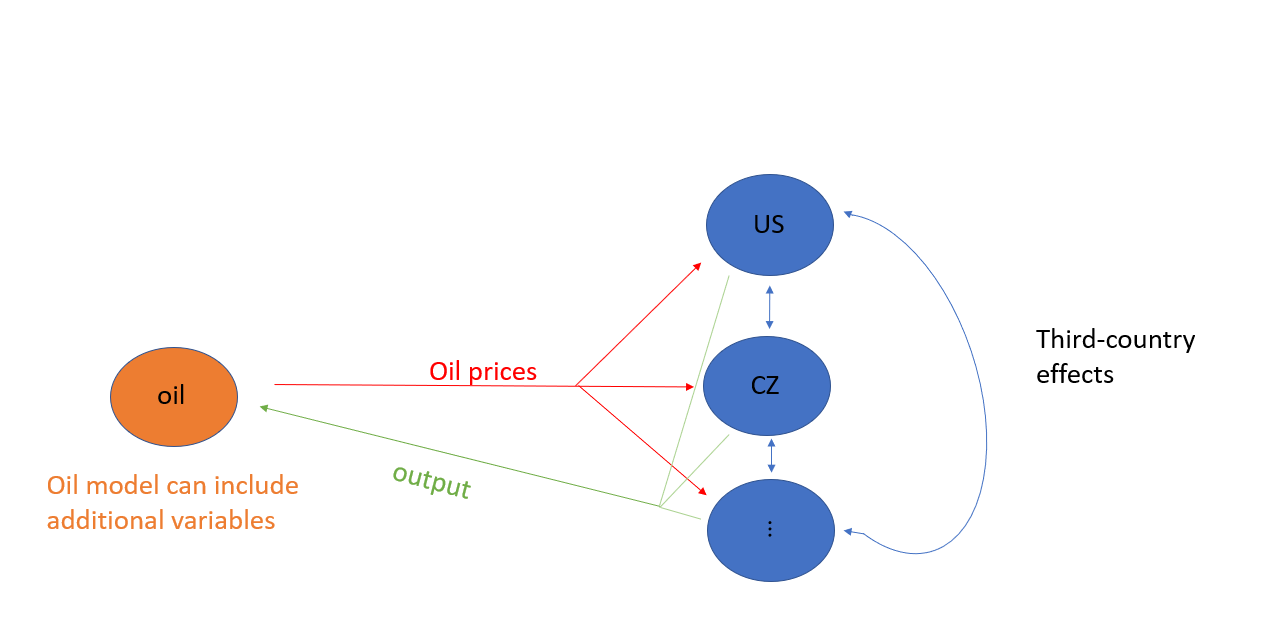“GVAR with oil prices modeled separately.”

For that purpose we have to remove oil prices from the US model and attach them to a separate slot in the data list. This slot has to have its own country label. We use ‘OC’ for “oil country”.

eerData2<-eerData
eerData2$OC<-eerData$US[,c("poil"),drop=FALSE] # move oil prices into own slot
eerData2$US<-eerData$US[,c("y","Dp", "rer" , "stir", "ltir","tb")] # exclude it from US m odel

Now we have to specify a list object that we label OC.weights. The list has to consist of three slots with the following names weights, variables and exo:

OC.weights<-list()
OC.weights$weights<-rep(1/3, 3) names(OC.weights$weights)<-names(eerData2)[1:3] # last one is OC model, hence only until 3
OC.weights$variables<-c(colnames(eerData2$OC),"y") # first entry, endog. variables, second entry weighted average of y from the other countries to proxy demand
OC.weights$exo<-"poil" The first slot, weights, should be a vector of weights that sum up to unity. In the example above, we simply use $$1/N$$, other weights could include purchasing power parities (PPP). The weights are used to aggregate specific variables that in turn enter the oil model as weakly exogenous. The second slot, variables, should specify the names of the endogenous and weakly exogenous variables that are used in the OC model. In the oil price example, we include the oil price (poil) as an endogenous variable (not contained in any other country model) and a weighted average using weights of output (y) to proxy world demand as weakly exogenous variable. Next, we specify via exo which one of the endogenous variables of the OC model are fed back into the other country models. In this example we specify poil. Last, we put all this information in a further list called OE.weights (other entity weights). This is done to allow for multiple other entity models (i.e., an oil price model, a joint monetary union model, etc.). It is important that the list entry has the same name as the other entity model, in our example OC. # other entities weights with same name as new oil country OE.weights <- list(OC=OC.weights) Now we can re-estimate the model where we pass on OE.weights via the expert argument. model.ssvs.4<-bgvar(Data=eerData2, W=W.trade0012, plag=1, draws=100, burnin=100, prior="SSVS", SV=TRUE, expert=list(OE.weights=OE.weights, save.shrink.store=TRUE), trend=TRUE ) and can compare the results of the four models by e.g., looking at the average PIPs. aux1<-model.ssvs.1$cc.results$PIP$PIP.avg;aux1<-aux1[-nrow(aux1),1:6]
aux2<-model.ssvs.2$cc.results$PIP$PIP.avg;aux2<-aux2[-nrow(aux2),1:6] aux3<-model.ssvs.3$cc.results$PIP$PIP.avg;aux3<-aux3[-nrow(aux3),1:6]
aux4<-model.ssvs.4$cc.results$PIP$PIP.avg;aux4<-aux4[-nrow(aux4),1:6] heatmap(aux1,Rowv=NA,Colv=NA, main="Model 1") heatmap(aux2,Rowv=NA,Colv=NA, main="Model 2") heatmap(aux3,Rowv=NA,Colv=NA, main="Model 3") heatmap(aux4,Rowv=NA,Colv=NA, main="Model 4")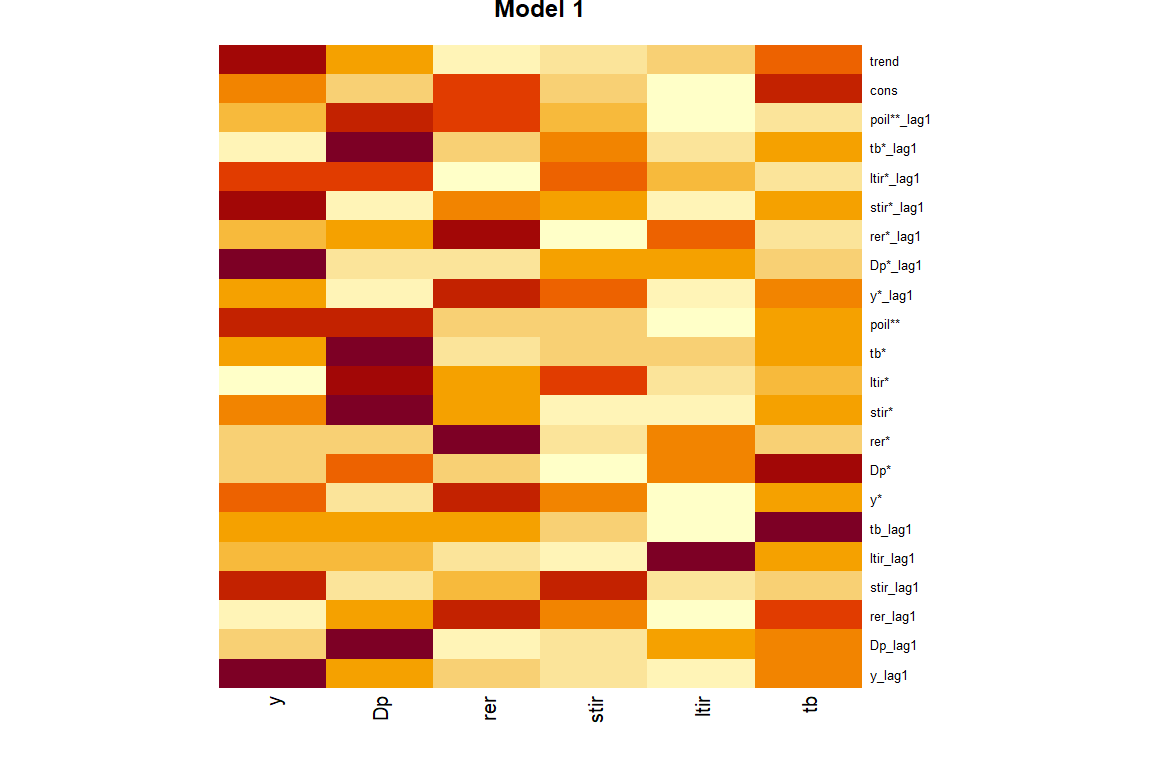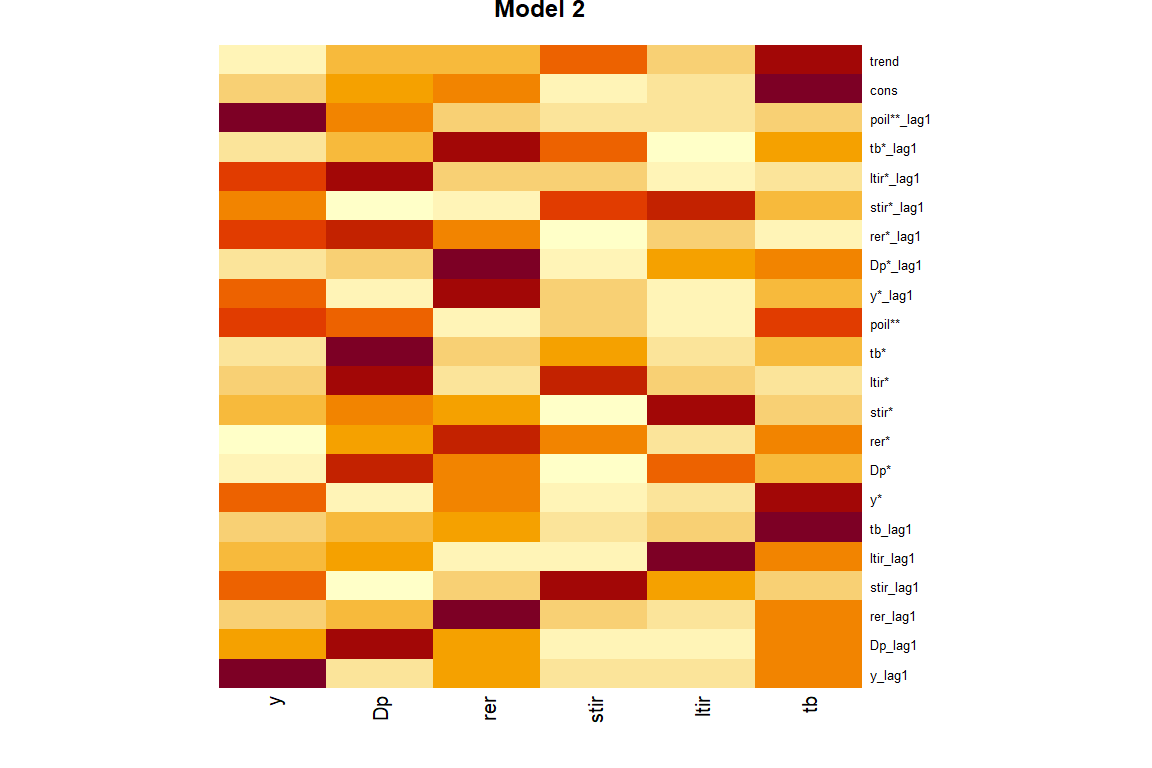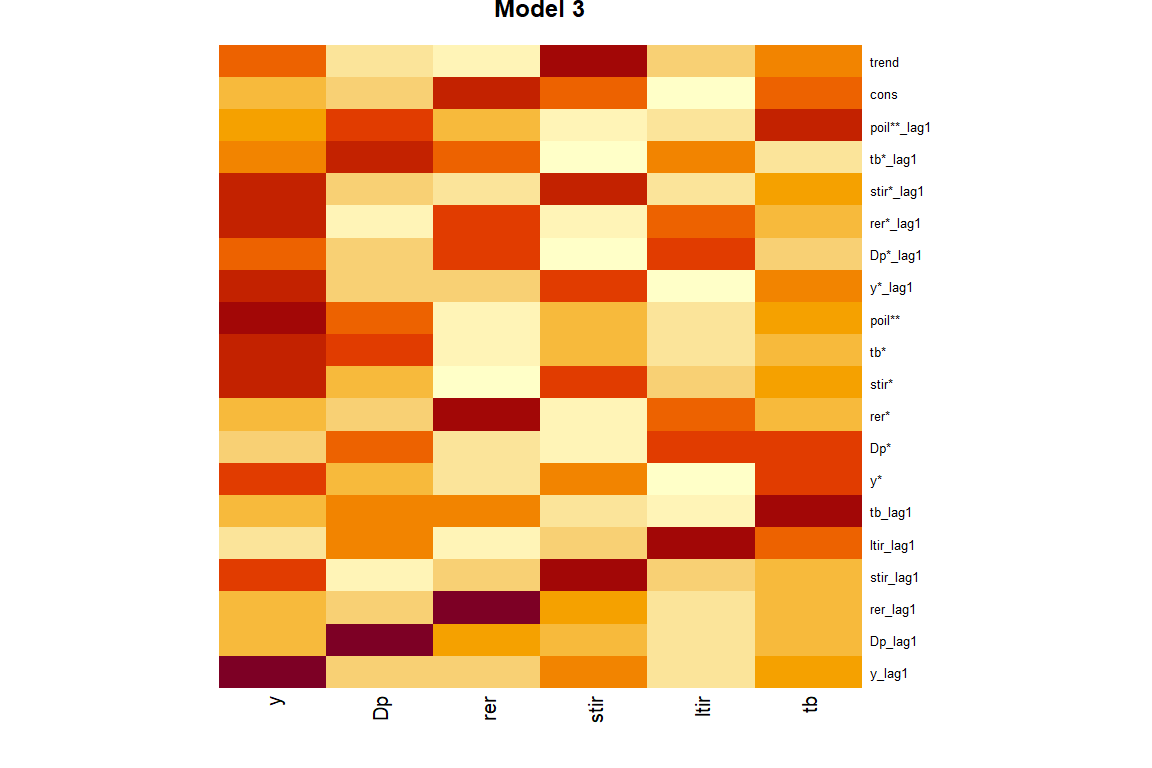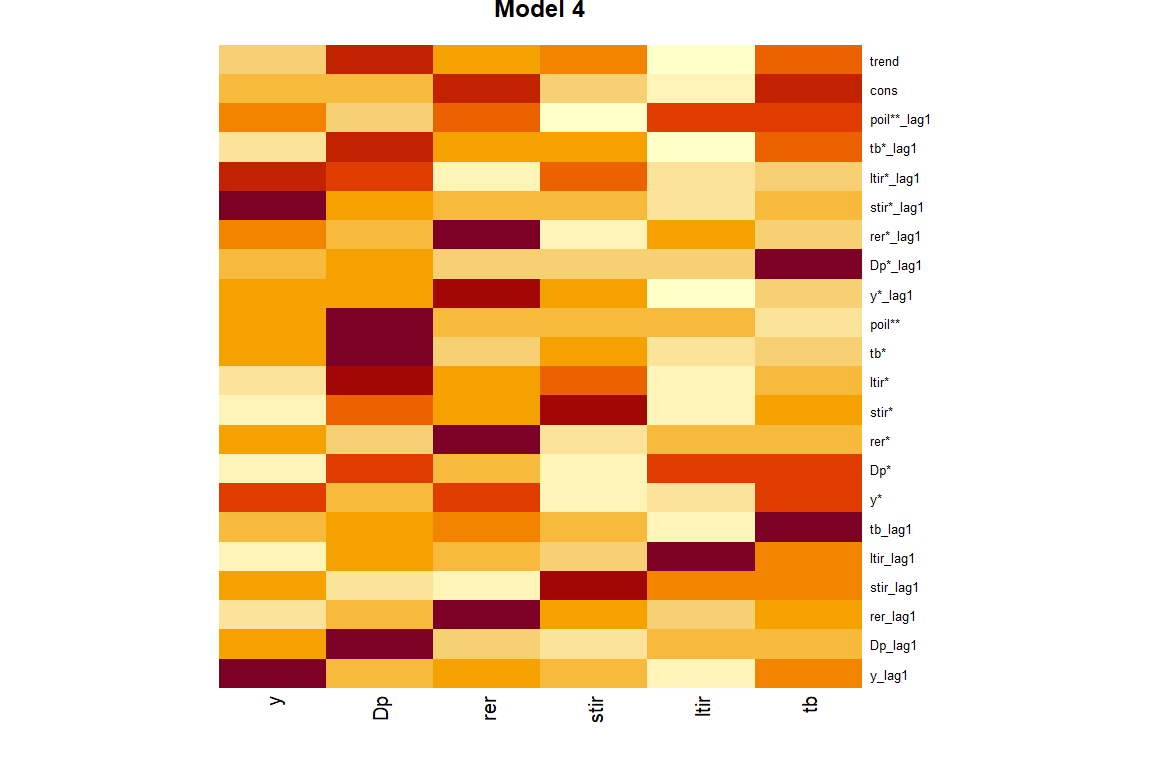Heatmaps of PIPs. We could also compare the models based on their fit, the likelihood, information criteria such as the DIC, residual properties or their forecasting performance. # Impulse response functions The package allows to calculate three different ways of dynamic responses, namely generalized impulse response functions (GIRFs) as in Pesaran and Shin (1998), orthogonalized impulse response functions using a Cholesky decomposition of the variance covariance matrix and finally impulse response functions given a set of user-specified sign restrictions. ## Recursive Identification and GIRFs Most of the GVAR applications deal with locally identified shocks. This implies that the shock of interest is orthogonal to the other shocks in the same unit model and hence can be interpreted in a structural way. There is still correlation between the shocks of the unit models, and these responses (the spillovers) are hence not fully structural (Eickmeier and Ng 2015). Hence some GVAR applications favor generalized impulse response functions, which per se do not rely on an orthogonalization. In BGVAR, responses to both types of shocks can be easily analyzed using the irf function. This function needs as input a model object (x), the impulse response horizon (n.ahead) and the default identification method is the recursive identification scheme via the Cholesky decomposition. Further arguments can be passed on using the wrapper expert and are discussed in the helpfiles. The following provides impulse response to all N shocks with unit scaling and using generalized impulse response functions: irf.chol<-irf(model.ssvs.1, n.ahead=24, expert=list(save.store=FALSE)) The results are stored in irf.chol$posterior, which is a four-dimensional array: $$K \times n.ahead \times nr.of shocks \times Q$$, with Q referring to the 50%, 68% and 95% quantiles of the posterior distribution of the impulse response functions. The posterior median of responses to the first shock could be accessed via irf.girf$posterior[,,1,"Q50"] Note that this example was for illustrational purposes; in most instances, we would be interested in a particular shock and calculating responses to all shocks in the system is rather inefficient. Hence, we can provide the irf function with more information. To be more precise, let us assume that we are interested in an expansionary monetary policy shock (i.e., a decrease in short-term interest rates) in the US country model. For that purpose, we can set up an shockinfo object, which contains information about which variable we want to shock (shock), the size of the shock (scale), the specific identification method(ident), and whether it is a shock applied in a single country or in multiple countries (global). We can use the helper function get_shockinfo() to set up a such a dummy object which we can subsequently modify according to our needs. The following lines of code are used for a negative 100 bp shock applied to US short term interest rates: # US monetary policy shock - Cholesky shockinfo_chol<-get_shockinfo("chol") shockinfo_chol$shock<-"US.stir"
shockinfo_chol$scale<--100 # US monetary policy shock - GIRF shockinfo_girf<-get_shockinfo("girf") shockinfo_girf$shock<-"US.stir"
shockinfo_girf$scale<--100 The shockinfo objects for Cholesky and GIRFs look exactly the same but have additionally an attribute which classifies the particular identification scheme. If we compare them, we notice that both have three columns defining the shock, the scale and whether it is defined as global shock. But we also see that the attributes differ which is important for the identification in the irf function. shockinfo_chol ## shock scale global ## 1 US.stir -100 FALSE shockinfo_girf ## shock scale global ## 1 US.stir -100 FALSE Now, we identify a monetary policy shock with recursive identification: irf.chol.us.mp<-irf(model.ssvs.1, n.ahead=24, shockinfo=shockinfo_chol, expert=list(save.store=TRUE)) The results are stored in irf.chol.us.mp. In order to save the complete set of draws, one can activate the save.store argument by setting it to TRUE within the expert settings (note: this may need a lot of storage). names(irf.chol.us.mp) ##  "posterior" "ident" "shockinfo" "rot.nr" "struc.obj" "model.obj" ##  "IRF_store" Again, irf.chol.us.mp$posterior is a $$K \times n.ahead \times nr.of shocks \times 7$$ object and the last slot contains the 50%, 68% and 95% credible intervals along with the posterior median. If save.store=TRUE, IRF_store contains the full set of impulse response draws and you can calculate additional quantiles of interest.

We can plot the complete responses of a particular country by typing:

plot(irf.chol.us.mp, resp="US", shock="US.stir")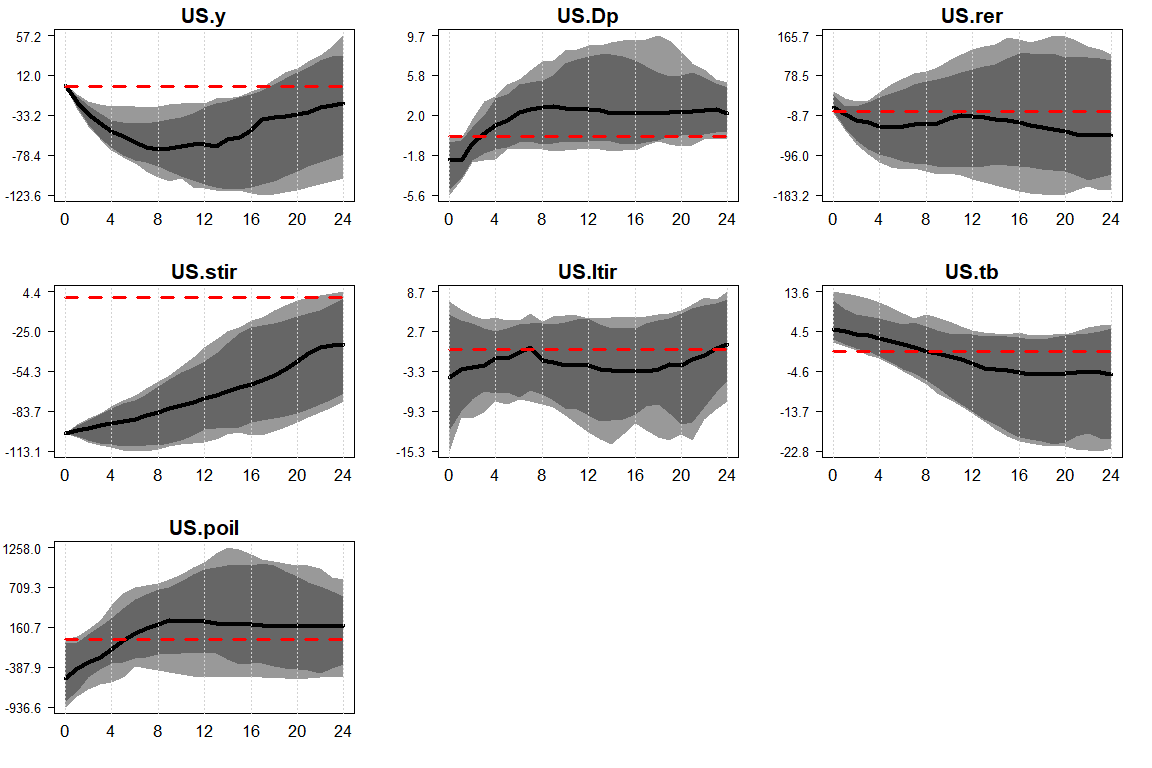Responses of US country model

The plot shows the posterior median response (solid, black line) along 50% (dark grey) and 68% (light grey) credible intervals.

We can also compare the Cholesky responses with GIRFs. For that purpose, let us look at a GDP shock.

# cholesky
shockinfo_chol       <- get_shockinfo("chol", nr_rows = 2)
shockinfo_chol$shock <- c("US.stir","US.y") shockinfo_chol$scale <- c(1,1)
# generalized impulse responses
shockinfo_girf       <- get_shockinfo("girf", nr_rows = 2)
shockinfo_girf$shock <- c("US.stir","US.y") shockinfo_girf$scale <- c(1,1)
# Recursive US GDP
# GIRF US GDP
irf.girf.us.y<-irf(model.ssvs.1, n.ahead=24, shockinfo=shockinfo_girf)
plot(irf.chol.us.y, resp="US.y", shock="US.y")
plot(irf.girf.us.y, resp="US.y", shock="US.y")
plot(irf.chol.us.y, resp="US.rer", shock="US.y")
plot(irf.girf.us.y, resp="US.rer", shock="US.y")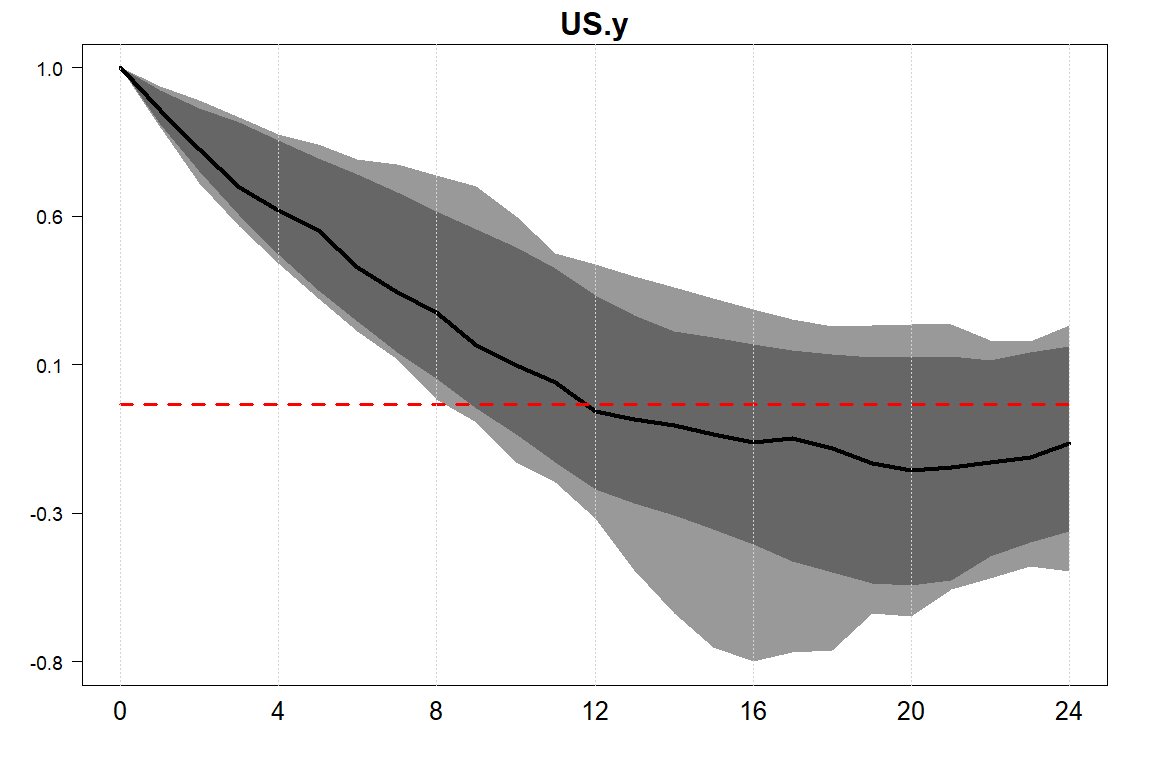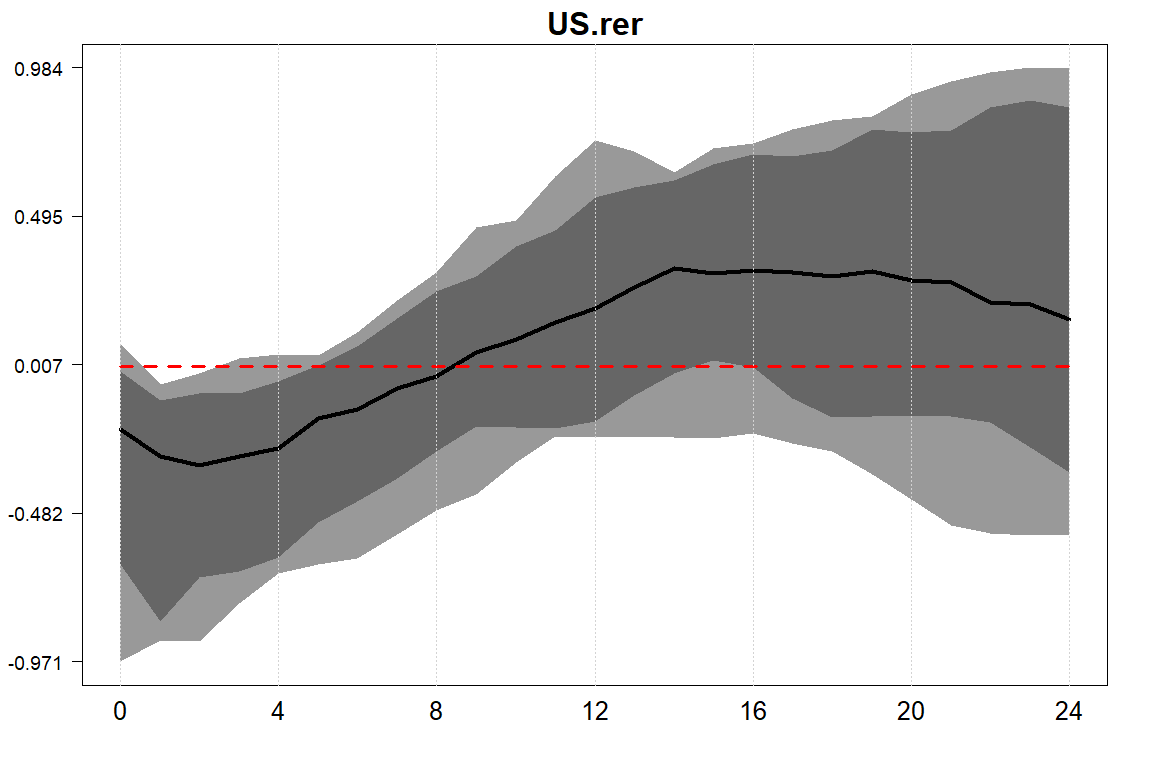Comparison of responses Cholesky (left) and GIRF (right) to a negative GDP shock.

We see that the responses are similar. This is not surprising because we have shocked the first variable in the US country model (y) and there are no timing restrictions on the remaining variables (they are all affected without any lag). In that case, the orthogonal impulse responses and the GIRF coincide.

Last, we could also look at a joint or global shock. For example, we could be interested in the effects of a simultaneous decrease in output across major economies, such as the G-7 and Russia. For that purpose, we have to set global<-TRUE. The following lines illustrate the joint GDP shock:

shockinfo<-get_shockinfo("girf", nr_rows = 3)
shockinfo$shock<-c("EA.y","US.y","RU.y") shockinfo$global<-TRUE
shockinfo$scale<--1 irf.global<-irf(model.ssvs.1, n.ahead=24, shockinfo=shockinfo) plot(irf.global, resp=c("US.y","EA.y","RU.y"), shock="Global.y")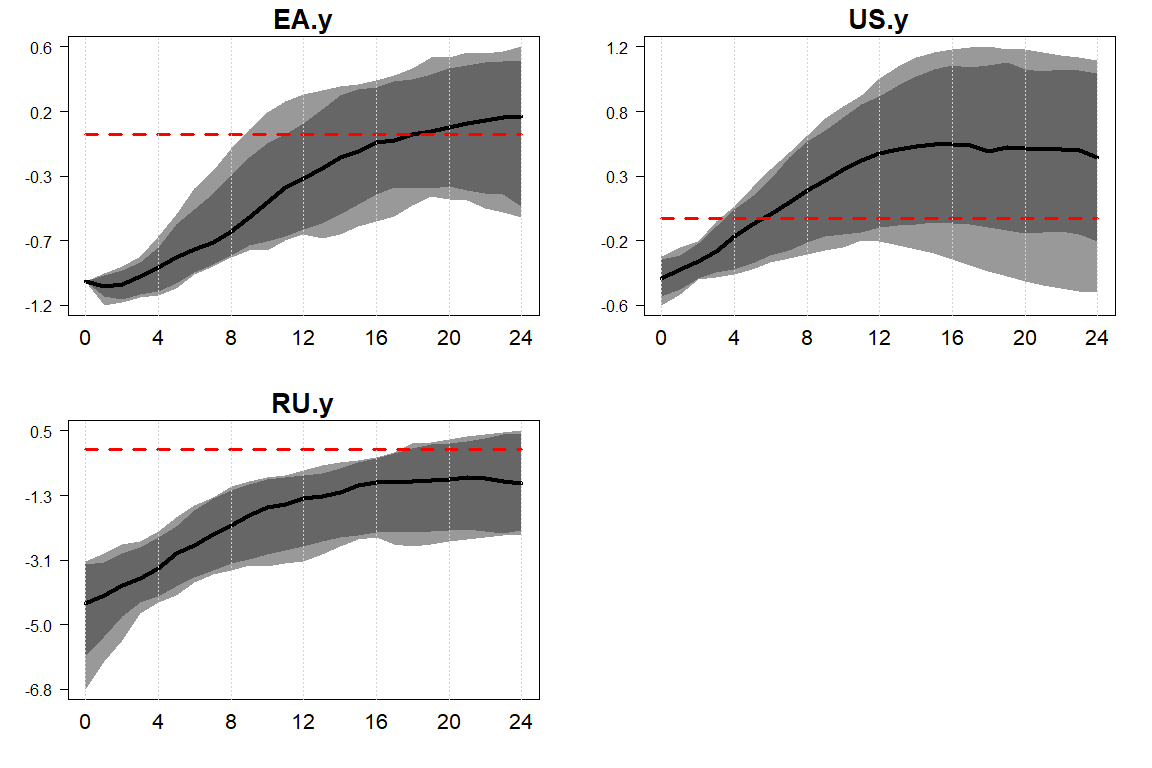## Identification with Zero- and Sign-Restrictions In this section, we identify the shocks locally with sign-restrictions. For that purpose, we will use another example data set and estimate a new GVAR. This data set contains one-year ahead GDP, inflation and short-term interest rate forecasts for the USA. The forecasts are from the survey of professional forecasters (SPF) data base. data("eerData") eerData<-eerData[cN] W.trade0012<-W.trade0012[cN,cN] W.trade0012<-apply(W.trade0012,2,function(x)x/rowSums(W.trade0012)) # append expectations data to US model temp <- cbind(USexpectations, eerData$US)
colnames(temp) <- c(colnames(USexpectations),colnames(eerData$US)) eerData$US <- temp
model.ssvs.eer<-bgvar(Data=eerData,
plag=1,
draws=100,
burnin=100,
prior="SSVS",
SV=TRUE)

For now, we start with an identification of two standard shocks in economics in the US model, namely an aggregate demand and aggregate supply shock. While the shockinfo was optional when using Cholesky / GIRFs, it is mandatory when working with sign restrictions. We do this in two steps, first we create a dummy object with get_shockinfo("sign") that contains information on the general shock setting and then add sign restrictions one-by-one using add_shockinfo(). The following illustrates this:

shockinfo<-get_shockinfo("sign")
restriction="US.Dp", sign=">", horizon=1, prob=1, scale=1)
restriction="US.y", sign="<", horizon=1, prob=1, scale=1)

In add_shockinfo we provide information on which variable to shock (shock), on which responses to put the sign restrictions (restriction), the direction of the restriction (sign) and the horizon how long these restrictions should hold (horizon). Note that the shock is always positive, but can be re-scaled by scale. The argument prob allows you to specify a percentage of the draws for which the restrictions have to hold. This argument might be useful when working with cross-sectional sign restrictions, where the idea is that some restrictions have to hold on average or at a certain percentage. The default is prob=1. If we want to add more restrictions to a particular shock, we can simply provide a vector instead of a scalar add_shockinfo(shockinfo, shock="US.Dp",restriction=c("US.y", "US.stir"), sign=c("<","<"), horizon=c(1,1), prob=c(1,1), scale=1) Note that increasing the number of restrictions (on the variables or the horizon) will lead to more precise inference; however, finding a suitable rotation matrix will become substantially harder.

We then invoke the irf() command to compute the impulse responses. The function draws rotation matrices using the algorithm of Rubio-Ramirez, Waggoner, and Zha (2010). In case we specify additional zero restrictions (see the next example below), we use the algorithm of Arias, Rubio-Ramirez, and Waggoner (2018). By default, we use one CPU core (cores=NULL) and do not store the full set of responses (save.store=FALSE). The maximum number of rotation matrices sampled per MCMC draw before we jump to the next draw can be specified by MaxTries.

irf.sign<-irf(model.ssvs.eer, n.ahead=24, shockinfo=shockinfo,
expert=list(MaxTries=100, save.store=FALSE, cores=NULL))

We can infer the number of successful rotation matrices by looking at

irf.sign$rot.nr ##  "For 46 draws out of 71 draws, a rotation matrix has been found." plot(irf.sign, resp=c("US.y","US.Dp"), shock="US.y") plot(irf.sign, resp=c("US.y","US.Dp"), shock="US.Dp")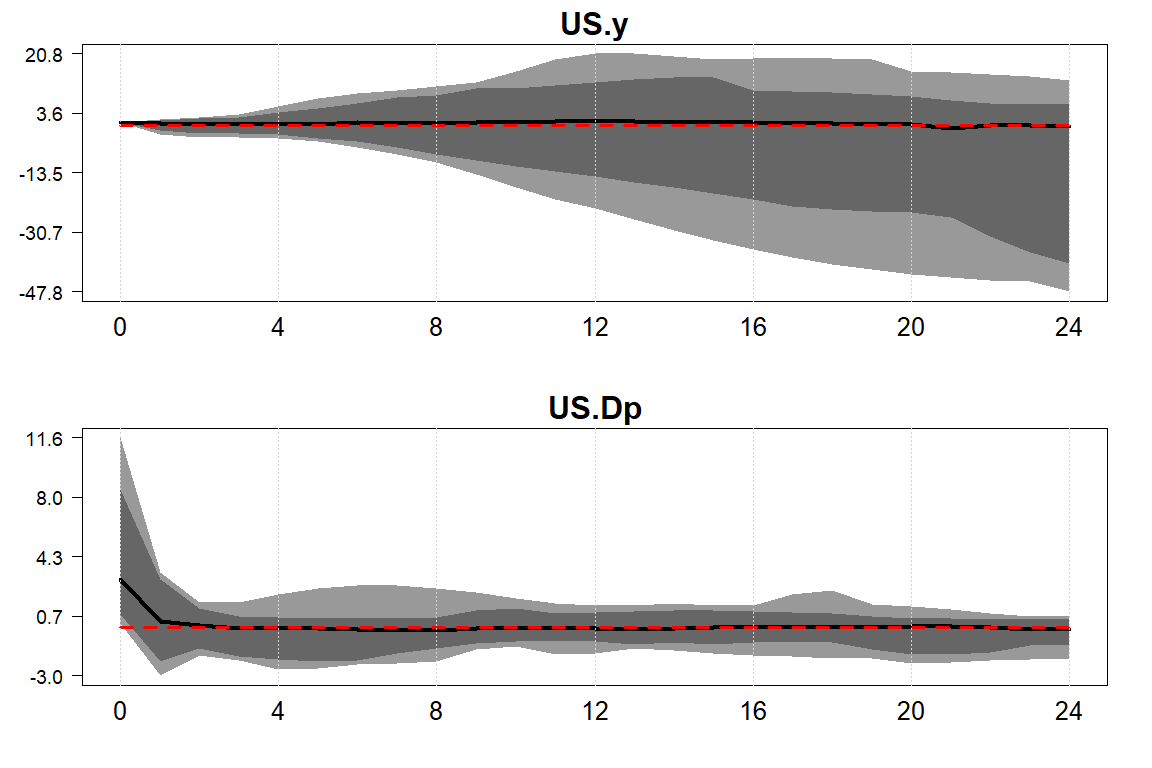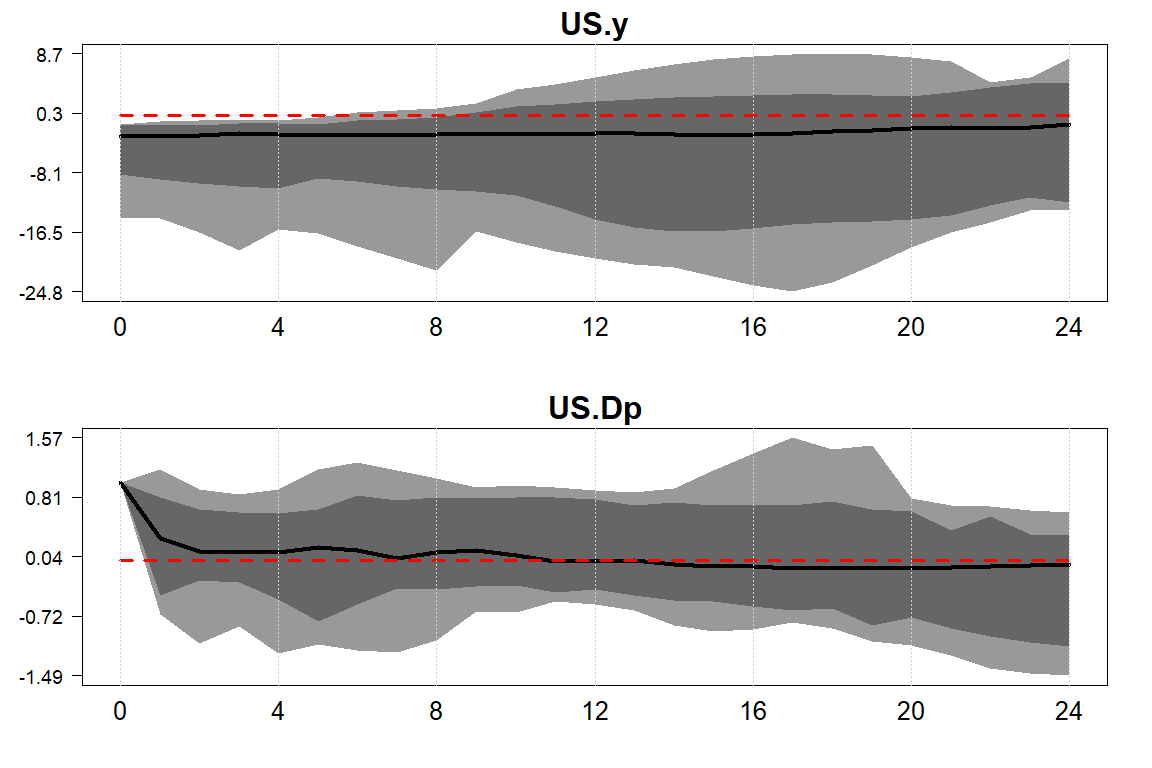Responses to AS (upper panel) and AD (lower panel) shock. Several recent papers advocate the inclusion of survey data in a VAR. Castelnuovo and Surico (2010) show that including inflation expectations mitigates the price puzzle (i.e., the counter intuitive positive movement of inflation in response to a monetary tightening). D’Amico and King (2015) go one step further and argue that expectations should always be included in a VAR model since they contain information that is not contained in standard macroeconomic data. They also show how to make inference with survey data in a VAR framework and propose so-called rationality conditions. For an application in a GVAR context, see Boeck, Feldkircher, and Siklos (2021). In a nutshell, these conditions put restrictions on actual data to match the expectations either on average over (ratio.average) or at the end of (ratio.H) the forecast horizon. Let us look at a concrete example. shockinfo<-get_shockinfo("sign") shockinfo<-add_shockinfo(shockinfo, shock="US.stir_t+4", restriction=c("US.Dp_t+4","US.stir","US.y_t+4","US.stir_t+4","US.Dp_t+4","US.y_t+4"), sign=c("<","0","<","ratio.avg","ratio.H","ratio.H"), horizon=c(1,1,1,5,5,5), prob=1, scale=1) irf.sign.zero<-irf(model.ssvs.eer, n.ahead=20, shockinfo=shockinfo, expert=list(MaxTries=100, save.store=TRUE)) The figure below shows the results for short term interest rates (stir) and output (y). # rationality condition: US.stir_t+4 on impact is equal to average of IRF of # US.stir between horizon 2 and 5 matplot(cbind(irf.sign.zero$IRF_store["US.stir_t+4",1,,1],
irf.sign.zero$IRF_store["US.stir",1,,1]), type="l",ylab="",main="stir",lwd=2,xaxt="n"); axis(side=1,at=c(1:5,9,13,17,21,25),label=c(0:4,8,12,16,20,24)) legend("topright",lty=c(1,2),c("expected","actual"),lwd=2,bty="n",col=c("black","red")) segments(x0=2,y0=1,x1=5,y1=1,lwd=2,lty=3,col="grey") points(1,1,col="grey",pch=19,lwd=4) abline(v=c(2,5),lty=3,col="grey",lwd=2) # rationality condition: US.y_t+4 on impact is equal to H-step ahead IRF # of US.y in horizon 5 matplot(cbind(irf.sign.zero$IRF_store["US.y_t+4",1,,1],
irf.sign.zero$IRF_store["US.y",1,,1]), type="l",ylab="",main="y",lwd=2,xaxt="n") axis(side=1,at=c(1:5,9,13,17,21,25),label=c(0:4,8,12,16,20,24)) legend("topright",lty=c(1,2),c("expected","actual"),lwd=2,bty="n",col=c("black","red")) yy<-irf.sign.zero$IRF_store["US.y_t+4",1,1,1]
segments(x0=1,y0=yy,x1=5,y1=yy,lwd=2,lty=3,col="grey");abline(v=c(1,5),col="grey",lty=3)
points(1,yy,col="grey",pch=19,lwd=4);points(5,yy,col="grey",pch=19,lwd=4)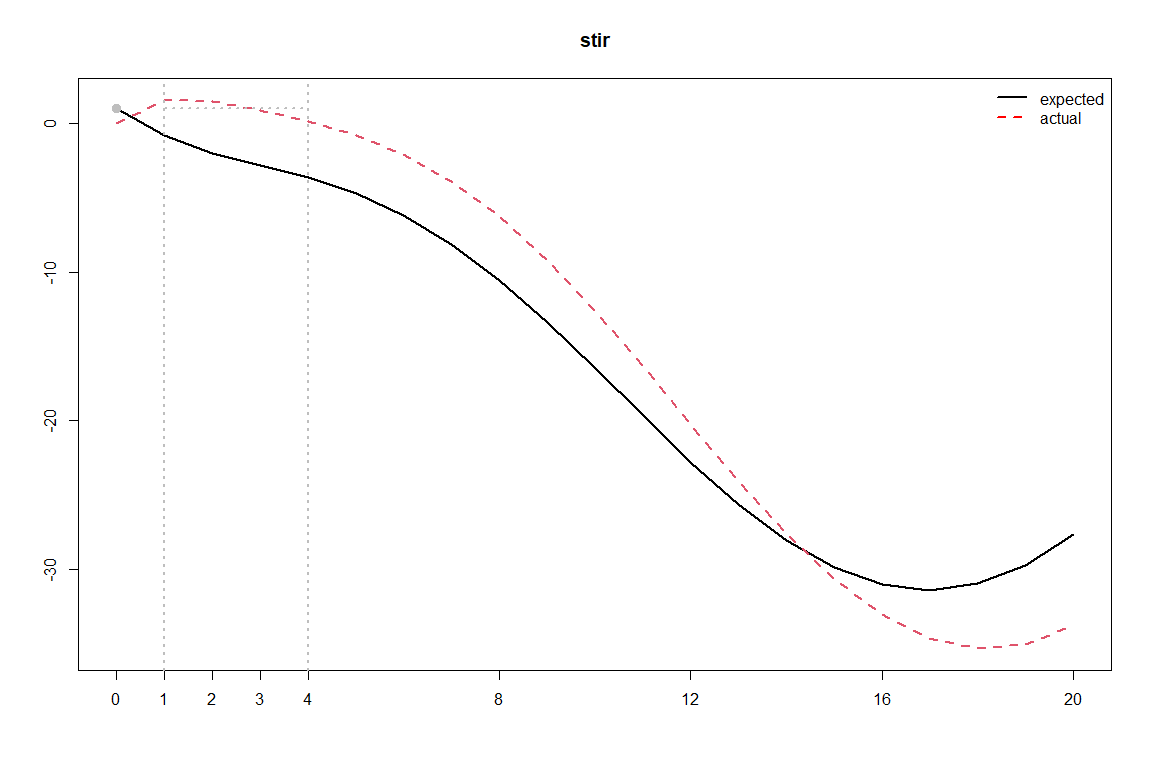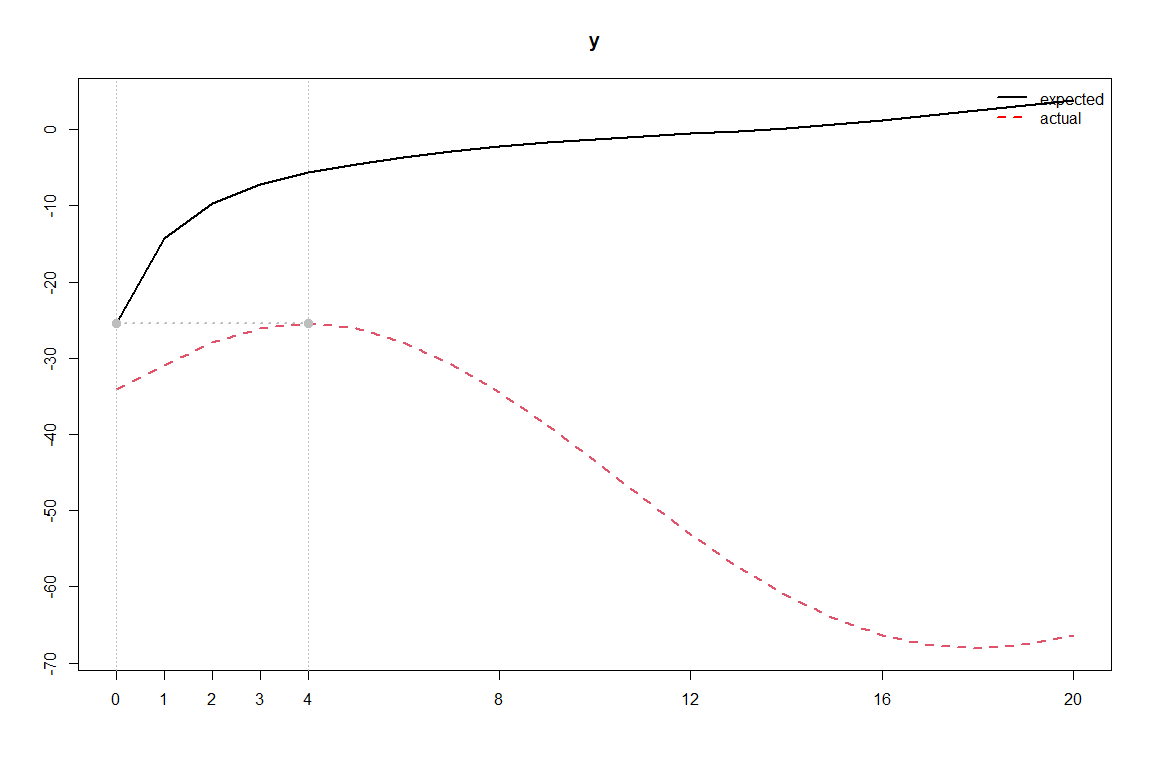Rationality conditions I.

Impulse responses that refer to observed data are in red (dashed), and the ones referring to expected data in black. The condition we have imposed on short-term interest rates (top panel) was that observed rates should equal the shock to expected rates on average over the forecast horizon (one year, i.e., on impact plus 4 quarters). The respective period is marked by the two vertical, grey lines. Put differently, the average of the red-dashed line over the forecast horizon has to equal the expectation shock on impact (grey dot).

On output, shown in the bottom panel, by contrast, we have imposed a condition that has to hold exactly at the forecast horizon. The red line, the impulse response of observed output, has to meet the impact response of expected output at $$h=5$$. In the figure, these two points are indicated by the two grey dots.

The last example we look at is how to put restrictions on the cross-section. Chudik and Fidora (2011) and Cashin et al. (2014) argue that a major advantage of GVARs is that they allow to put restrictions also on variables from different countries, which should further sharpen inference. They apply cross-sectional restrictions to identify oil supply and demand shocks with the restrictions on oil importing countries’ GDP.

Here, we follow Feldkircher, Gruber, and Huber (2020) who use cross-sectional restrictions to identify a term spread shock in the euro area. Since they use separate country models for members of the euro area, the joint monetary policy has to be modeled. One idea that has been put forth in recent applications is to set up an additional country for the joint monetary policy in the euro area. In the next example, we follow Georgiadis (2015) and set up a ECB model that determines euro area interest rates according to a Taylor rule. This idea follows the set-up of the additional oil price model and can be summarized graphically in the picture below.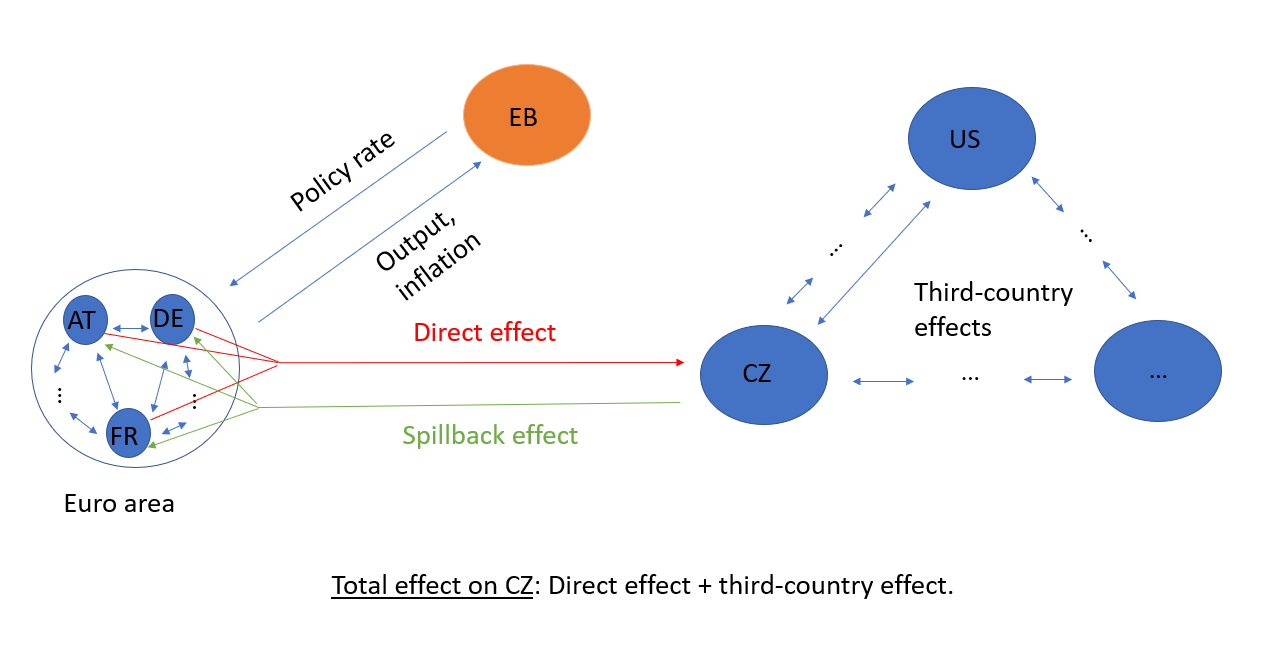“GVAR with euro area members modeled seperately.”

We can look at the data by typing:

data(monthlyData);monthlyData$OC<-NULL names(monthlyData) ##  "BG" "CZ" "DK" "HR" "HU" "PL" "RO" "SE" "GB" "AT" "BE" "DE" "GR" "ES" "FI" ##  "FR" "IE" "IT" "NL" "PT" "US" "CN" "JP" "RU" "TR" "CA" "EB" # list of weights of other entities with same name as additional country model OE.weights = list(EB=EB.weights) EA_countries <- c("AT", "BE", "DE","ES", "FI","FR") # "IE", "IT", "NL", "PT","GR","SK","MT","CY","EE","LT","LV") To estimate the GVAR with an ‘EB’ country model, we have to specify additional arguments similar to the example with the oil price model discussed above. The monthlyData set already comes along with a pre-specified list EA.weights with the mandatory slots weights, variables and exo. The specification implies that the euro area monetary policy model (EB) includes EAstir, total.assets, M3, ciss as endogenous variables (these are contained in monthlyData$EB). We use PPP-weights contained in weights to aggregate output (y) and prices (p) from euro area countries and include them as weakly exogenous variables. Euro area short-term interest rates (EAstir) and the ciss indicator (ciss), specified in exo, are then passed on as exogenous variables to the remaining countries. Finally, we put EA.weights into the OE.weights list and label the slot EB (as the name of the additional country model, names(monthlyData)) and estimate the model:

monthlyData <- monthlyData[c(EA_countries,"EB")]
W<-W[EA_countries,EA_countries]
W<-apply(W,2,function(x)x/rowSums(W))
OE.weights$EB$weights <- OE.weights$EB$weights[names(OE.weights$EB$weights)%in%EA_countries]
# estimates the model
model.ssvs<-bgvar(Data=monthlyData,
W=W,
draws=200,
burnin=200,
plag=1,
prior="SSVS",
eigen=1.05,
expert=list(OE.weights=OE.weights))
##
##
## Start estimation of Bayesian Global Vector Autoregression.
##
## Prior: Stochastic Search Variable Selection prior.
## Lag order: 1 (endo.), 1 (w. exog.)
## Stochastic volatility: enabled.
## Number of cores used: 1.
## Thinning factor: 1. This means every draw is saved.
## Hyperparameter setup:
##   No hyperparameters are chosen, default setting applied.
##
## Estimation of country models starts...
##
## Start estimation of Bayesian Global Vector Autoregression.
##
## Prior: Stochastic Search Variable Selection prior.
## Lag order: 1 (endo.), 1 (w. exog.)
## Stochastic volatility: enabled.
## Number of cores used: 1.
## Thinning factor: 1. This means every draw is saved.
##   No hyperparameters are chosen, default setting applied.
##
## Estimation of country models starts...
## Model:  1 / 7  done.
##
## Start estimation of Bayesian Global Vector Autoregression.
##
## Prior: Stochastic Search Variable Selection prior.
## Lag order: 1 (endo.), 1 (w. exog.)
## Stochastic volatility: enabled.
## Number of cores used: 1.
## Thinning factor: 1. This means every draw is saved.
##   No hyperparameters are chosen, default setting applied.
##
## Estimation of country models starts...
## Model:  2 / 7  done.
##
## Start estimation of Bayesian Global Vector Autoregression.
##
## Prior: Stochastic Search Variable Selection prior.
## Lag order: 1 (endo.), 1 (w. exog.)
## Stochastic volatility: enabled.
## Number of cores used: 1.
## Thinning factor: 1. This means every draw is saved.
##   No hyperparameters are chosen, default setting applied.
##
## Estimation of country models starts...
## Model:  3 / 7  done.
##
## Start estimation of Bayesian Global Vector Autoregression.
##
## Prior: Stochastic Search Variable Selection prior.
## Lag order: 1 (endo.), 1 (w. exog.)
## Stochastic volatility: enabled.
## Number of cores used: 1.
## Thinning factor: 1. This means every draw is saved.
##   No hyperparameters are chosen, default setting applied.
##
## Estimation of country models starts...
## Model:  4 / 7  done.
##
## Start estimation of Bayesian Global Vector Autoregression.
##
## Prior: Stochastic Search Variable Selection prior.
## Lag order: 1 (endo.), 1 (w. exog.)
## Stochastic volatility: enabled.
## Number of cores used: 1.
## Thinning factor: 1. This means every draw is saved.
##   No hyperparameters are chosen, default setting applied.
##
## Estimation of country models starts...
## Model:  5 / 7  done.
##
## Start estimation of Bayesian Global Vector Autoregression.
##
## Prior: Stochastic Search Variable Selection prior.
## Lag order: 1 (endo.), 1 (w. exog.)
## Stochastic volatility: enabled.
## Number of cores used: 1.
## Thinning factor: 1. This means every draw is saved.
##   No hyperparameters are chosen, default setting applied.
##
## Estimation of country models starts...
## Model:  6 / 7  done.
##
## Start estimation of Bayesian Global Vector Autoregression.
##
## Prior: Stochastic Search Variable Selection prior.
## Lag order: 1 (endo.), 1 (w. exog.)
## Stochastic volatility: enabled.
## Number of cores used: 1.
## Thinning factor: 1. This means every draw is saved.
##   No hyperparameters are chosen, default setting applied.
##
## Estimation of country models starts...
## Model:  7 / 7  done.
##
## Start estimation of Bayesian Global Vector Autoregression.
##
## Prior: Stochastic Search Variable Selection prior.
## Lag order: 1 (endo.), 1 (w. exog.)
## Stochastic volatility: enabled.
## Number of cores used: 1.
## Thinning factor: 1. This means every draw is saved.
##   No hyperparameters are chosen, default setting applied.
##
## Estimation of country models starts...
## Estimation done and took 0 mins 9 seconds.
## Start stacking:
##
## Stacking finished.
## Computation of BGVAR yields 199 (100%) draws (active trimming).
##  Needed time for estimation of bgvar: 0 mins 9 seconds.

We can now impose a joint shock on long-term interest rates for selected countries using sign restrictions on the cross section with the following lines of code:

# imposes sign restrictions on the cross-section and for a global shock
# (long-term interest rates)
shockinfo<-get_shockinfo("sign")
for(cc in c("AT","BE","FR")){
restriction=paste0(cc,c(".ip",".p")),
sign=c("<","<"), horizon=c(1,1),
prob=c(0.5,0.5), scale=c(-100,-100),
global=TRUE)
}

We can have a look at the restrictions by looking at the shockinfo object:

shockinfo
##     shock restriction sign horizon scale prob global
## 1 AT.ltir       AT.ip    <       1    -1  0.5   TRUE
## 2 AT.ltir        AT.p    <       1    -1  0.5   TRUE
## 3 BE.ltir       BE.ip    <       1    -1  0.5   TRUE
## 4 BE.ltir        BE.p    <       1    -1  0.5   TRUE
## 5 FR.ltir       FR.ip    <       1    -1  0.5   TRUE
## 6 FR.ltir        FR.p    <       1    -1  0.5   TRUE

Note the column prob. Here, we have specified that the restrictions have to hold only for half of the countries. We could make the restrictions stricter by increasing the percentage.

We can now compute the impulse responses using the same function as before.

irf.sign.ssvs<-irf(model.ssvs, n.ahead=24, shockinfo=shockinfo, expert=list(MaxTries=500))

To verify the sign restrictions, type:

irf.sign.ssvs$posterior[paste0(EA_countries[-c(3,12)],".ltir"),1,1,"Q50"] ## AT.ltir BE.ltir ES.ltir FI.ltir FR.ltir ## -1.0000000 -0.9933509 -0.9632447 -0.8812171 -1.0231577 irf.sign.ssvs$posterior[paste0(EA_countries,".ip"),1,1,"Q50"]
##        AT.ip        BE.ip        DE.ip        ES.ip        FI.ip        FR.ip
## -0.002559368 -0.043602492 -0.006863765 -0.004440010  0.001735155 -0.034581966
irf.sign.ssvs$posterior[paste0(EA_countries,".ip"),1,1,"Q50"] ## AT.ip BE.ip DE.ip ES.ip FI.ip FR.ip ## -0.002559368 -0.043602492 -0.006863765 -0.004440010 0.001735155 -0.034581966 The following plots the output responses for selected euro area countries. plot(irf.sign.ssvs, resp=c("AT.ip"), shock="Global.ltir") plot(irf.sign.ssvs, resp=c("BE.ip"), shock="Global.ltir") plot(irf.sign.ssvs, resp=c("DE.ip"), shock="Global.ltir") plot(irf.sign.ssvs, resp=c("ES.ip"), shock="Global.ltir")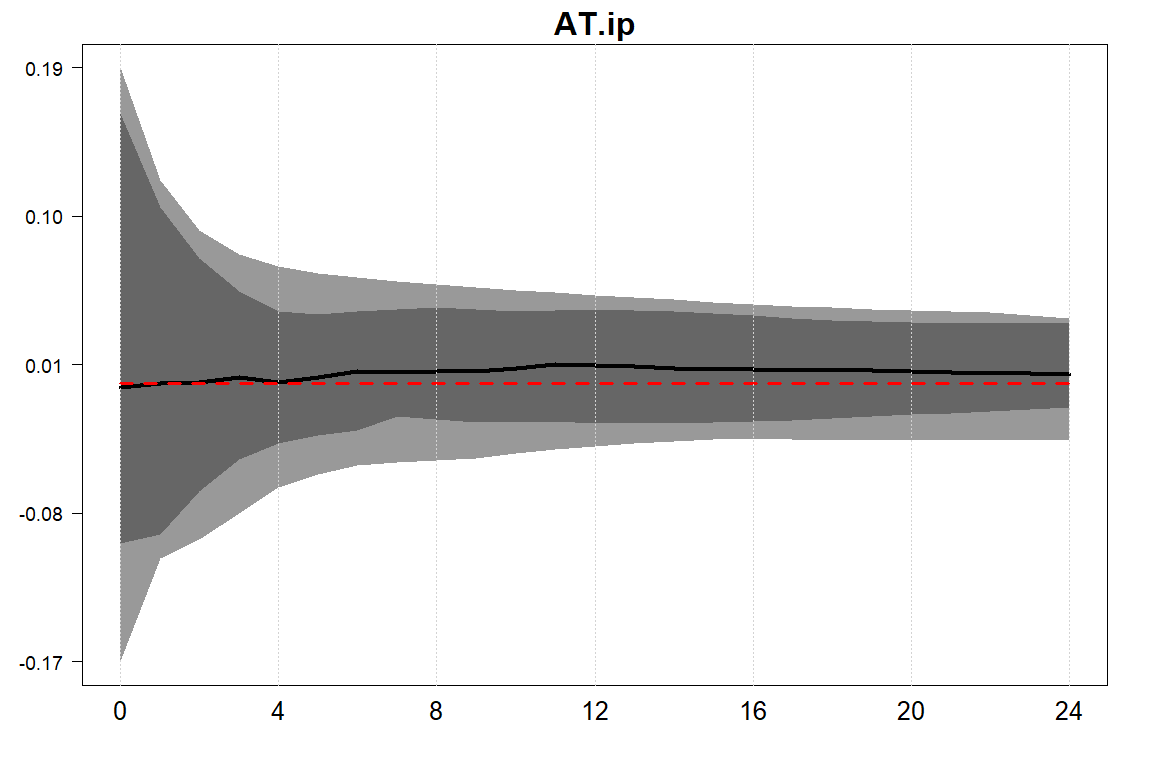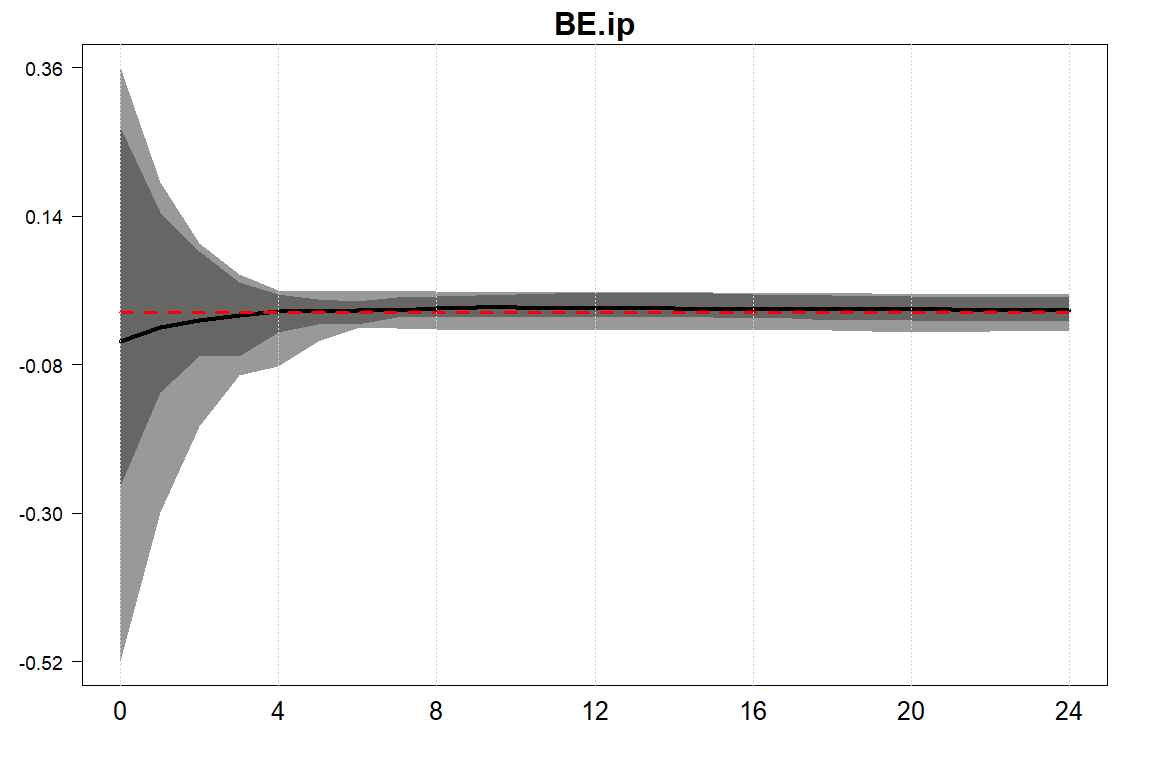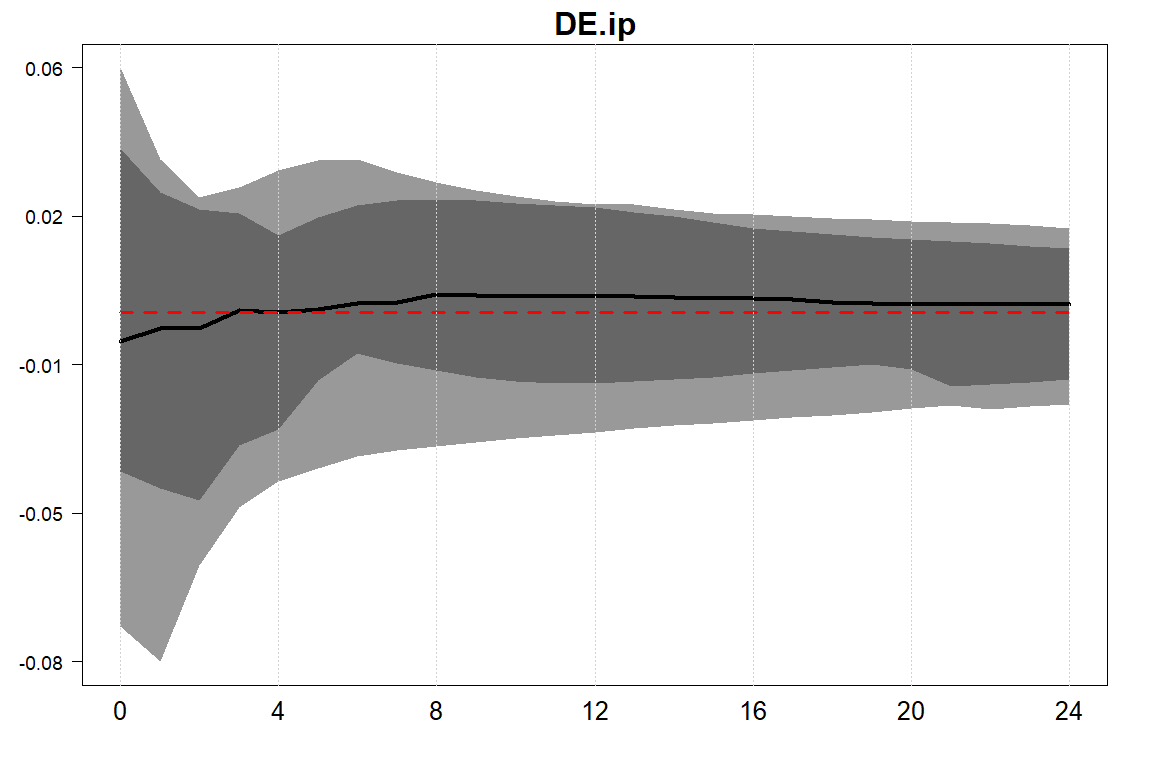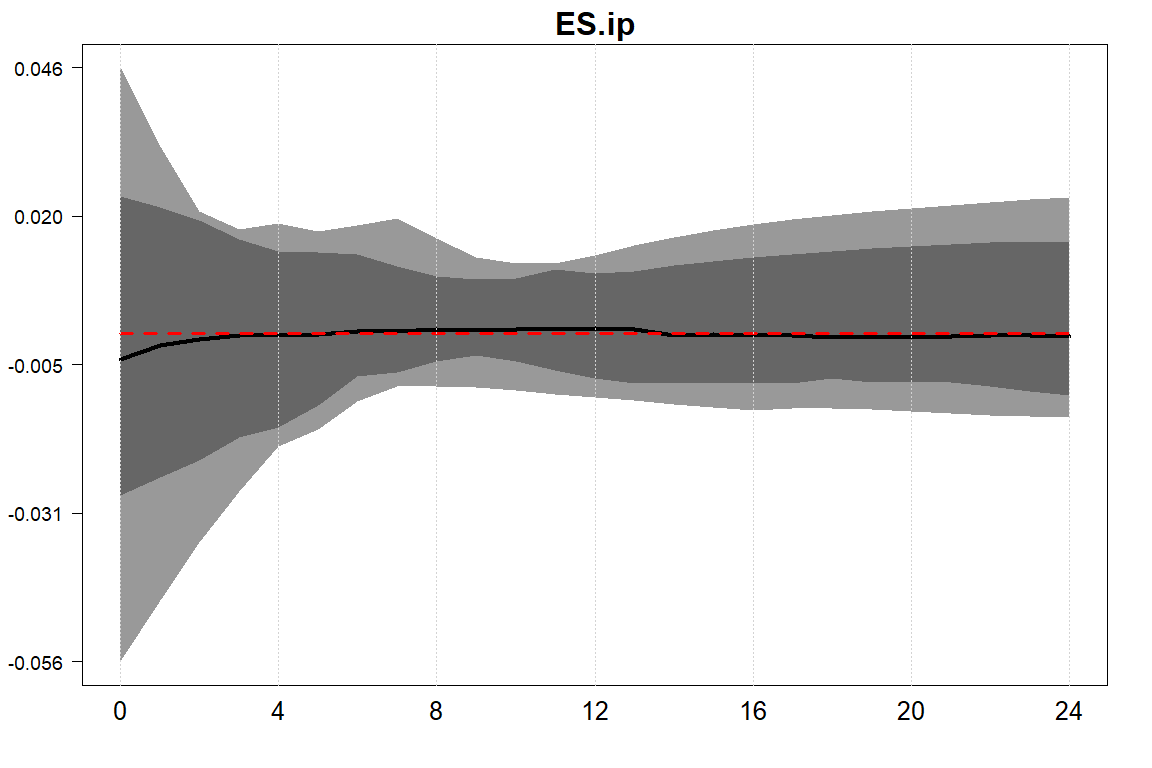Output responses of selected euro area countries. ## Forecast Error Variance Decomposition (FEVD) Forecast error variance decompositions indicate the amount of information each variable contributes to the other variables in the autoregression. It is calculated by examining how much of the forecast error variance of each of the variables can be explained by exogenous shocks to the other variables. In a system with fully orthogonalized errors, the shares of FEVD sum up to 1. In the GVAR context, however, since we identify a shock only locally in particular country model and we still have a certain degree of residual correlation, shares typically exceed unity. By contrast, a fully orthogonalized system obtained for example by means of a Cholesky decomposition would yield shares that sum up to unity but inherits assumptions that are probably hard to defend. In the case of the Cholesky decomposition, this would imply timing restrictions, i.e., which variables in which units are immediately affected or affected only with a lag. One way of fixing this is to use generalized forecast error variance decompositions. Like with GIRFs, these are independent of the ordering but, since the shocks are not orthogonalized, yield shares that exceed unity. Recently, Lanne and Nyberg (2016) proposed a way of scaling the GFEVDs, which has the nice property of shares summing up to 1 and results being independent of the ordering of the variables in the system. To calculate them, we can use the GFEVD.LN command. We can either use a running mean (running=TRUE) or the full set of posterior draws. The latter is computationally very expensive. #calculates the LN GFEVD gfevd.us.mp=gfevd(model.ssvs.eer,n.ahead=24,running=TRUE,cores=4)$FEVD
##
## Start computing generalized forecast error variance decomposition of Bayesian Global Vector Autoregression.
##
## Start computation on 4 cores (71 stable draws in total).
## Size of IRF object: 0.1 Mb
## Needed time for computation: 0 mins 2 seconds.
# get position of EA
idx<-which(grepl("EA.",dimnames(gfevd.us.mp)[]))
own<-colSums(gfevd.us.mp["EA.y",idx,])
foreign<-colSums(gfevd.us.mp["EA.y",-idx,])
barplot(t(cbind(own,foreign)),legend.text =c("own","foreign"))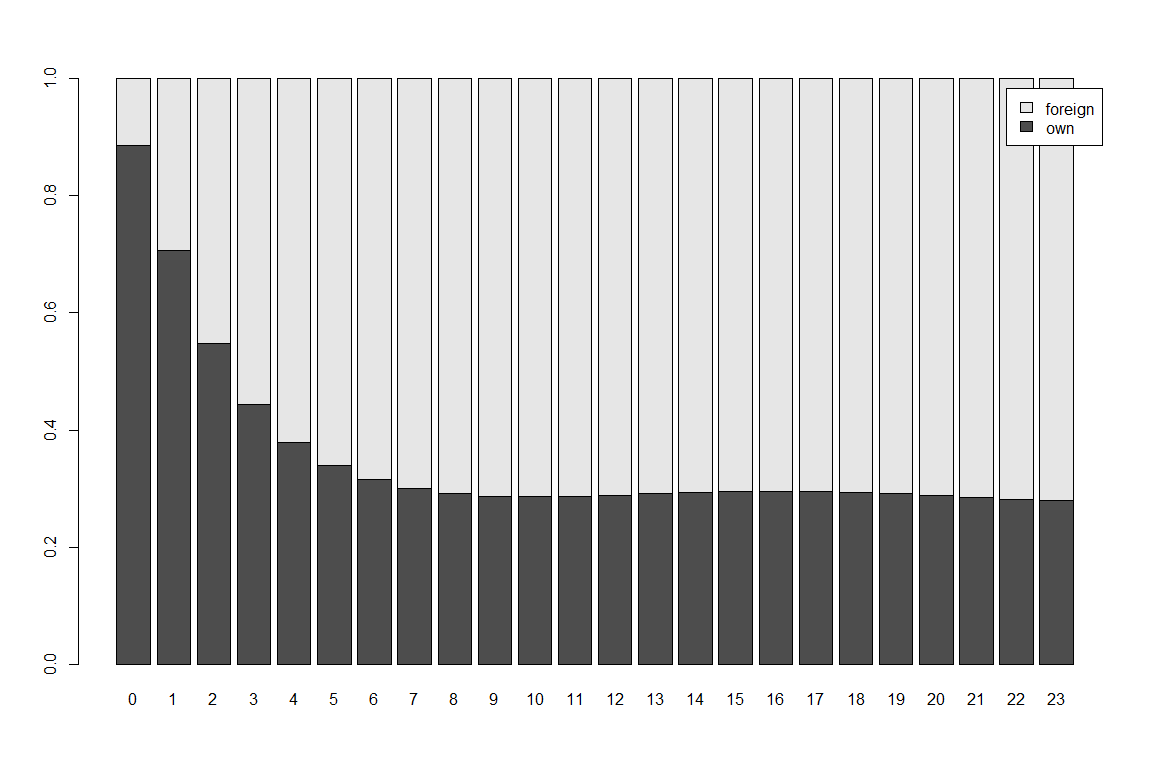FEVD of EA GDP.

The plot above shows a typical pattern: On impact and in the first periods, EA variables (own) explain a large share of GFEVD. With time and through the lag structure in the model, other countries’ variables show up more strongly as important determinants of EA output error variance.

In case we want to focus on a single country, which we have fully identified either using a Cholesky decomposition or sign restrictions, we can compute a simple forecast error variance decomposition (FEVD). This can be done by using the command fevd(). Since the computation is very time consuming, the FEVDs are based on the posterior median only (as opposed to calculating FEVDs for each MCMC draw or using a running mean). In case the underlying shock has been identified via sign restrictions, the corresponding rotation matrix is the one that fulfills the sign restrictions at the point estimate of the posterior median of the reduced form coefficients (stored in irf.obj$struc.obj$Rmed). Alternatively one can submit a rotation matrix using the option R.

# calculates FEVD for variables US.y
matplot(cbind(HD$x[,1],org.ts[,1]),type="l",ylab="",lwd=2) legend("bottomright",c("hd series","original"),col=c("black","red"),lty=c(1,2),bty="n",cex=2)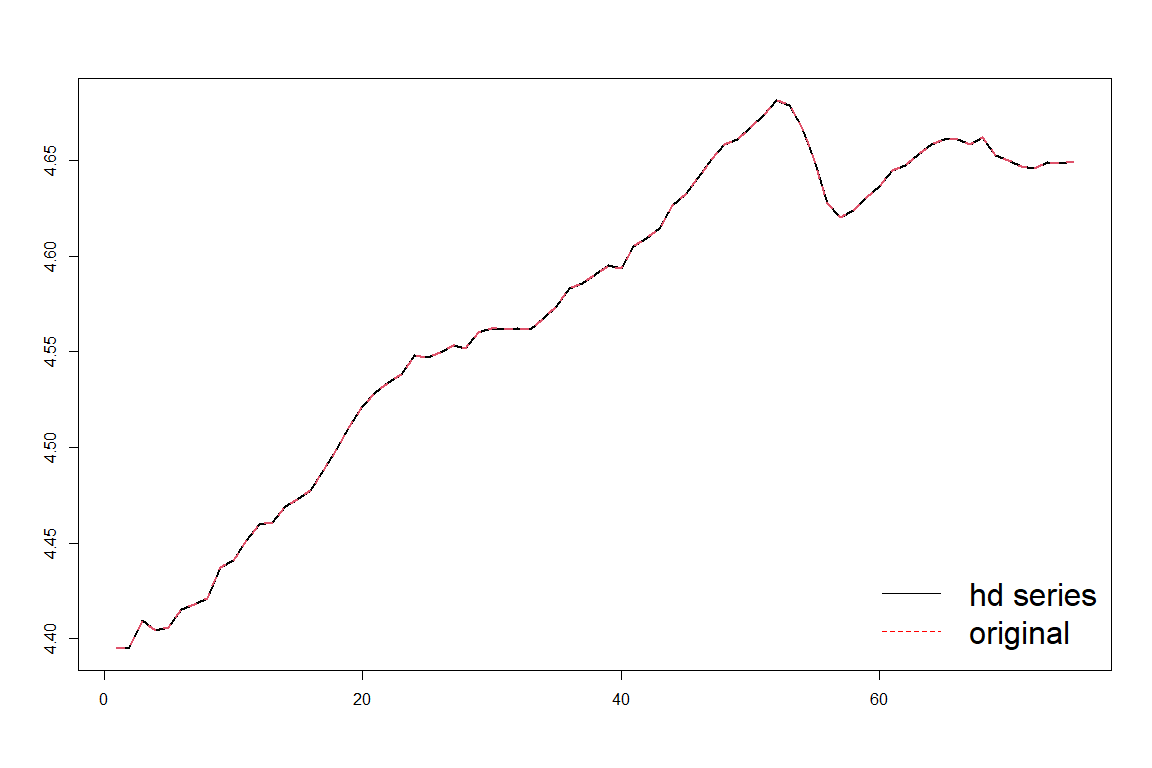Historical decomposition of euro area GDP. # Unconditional and Conditional Forecasts In this section, we demonstrate how the package can be used for forecasting. We distinguish between unconditional and conditional forecasting. Typical applications of unconditional forecasting are to select a model from a range of candidate models or for out-of-sample forecasting. Conditional forecasts can be used for scenario analysis by comparing a forecast with a fixed future path of a variable of interest to its unconditional forecast. ## Unconditional Forecasts Since the GVAR framework was developed to capture cross-country dependencies, it can handle a rich set of dynamics and interdependencies. This can also be useful for forecasting either global components (e.g., global output) or country-specific variables controlling for global factors. Pesaran, Schuermann, and Smith (2009) show that the GVAR yields competitive forecasts for a range of macroeconomic and financial variables. Crespo Cuaresma, Feldkircher, and Huber (2016) demonstrate that Bayesian shrinkage priors can help improving GVAR forecasts and Dovern, Feldkircher, and Huber (2016) and Huber (2016) yield evidence for further gains in forecast performance by using GVARs with stochastic volatility. To compute forecasts with the BGVAR package, we use the command predict. To be able to evaluate the forecast, we have to specify the size of the hold-out sample when estimating the model. Here, we choose a hold-out-sample of 8 observations by setting h=8 (the default values is h=0): model.ssvs.h8<-bgvar(Data=eerData, W=W.trade0012, draws=500, burnin=500, plag=1, prior="SSVS", hyperpara=NULL, SV=TRUE, thin=1, trend=TRUE, hold.out=8, eigen=1 ) The forecasts can then be calculated using the predict function. We calculate forecasts up to 8 periods ahead by setting n.ahead=8-step fcast <- predict(model.ssvs.h8, n.ahead=8, save.store=TRUE) The forecasts are stored in fcast$fcast which contains also the credible intervals of the predictive posterior distribution. We can evaluate the forecasts with the retained observations looking at the root mean squared errors (RMSEs) or log-predictive scores (LPS).

lps.h8 <- lps(fcast)
rmse.h8 <- rmse(fcast)

The objects lps.h8 and rmse.h8 then each contain a $$8 \times k$$ matrix with the LPS scores / RMSEs for each variable in the system over the forecast horizon.

Last, we can visualize the forecasts by typing

plot(fcast, resp="US.Dp", cut=8)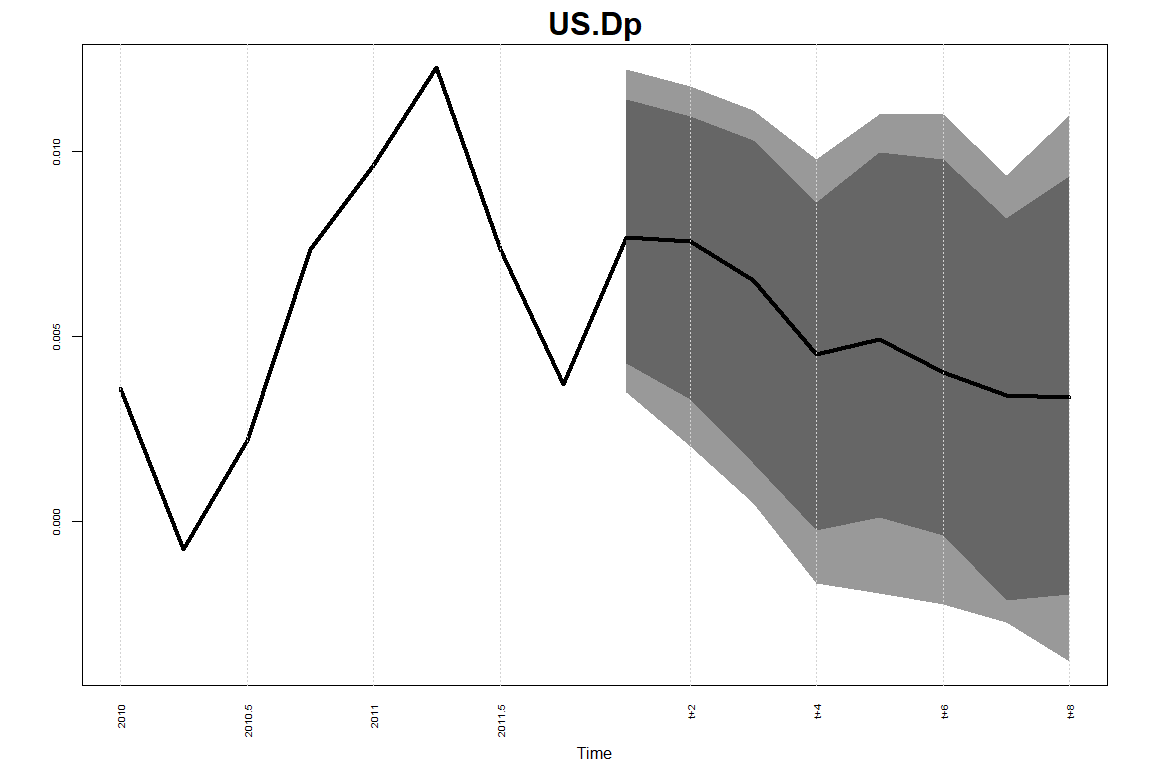Forecast plot.

with Cut denoting the number of realized data points that should be shown in the plot prior the forecasts start.

## Conditional Forecasts

Similar to structural analysis, it is possible to use conditional forecasts, identified in a country model. For that purpose, we use the methodology outlined in Waggoner and Zha (1999) and applied in Feldkircher, Huber, and Moder (2015) in the GVAR context. The following lines set up a conditional forecast holding inflation in the US country model fixed for five periods to its last observed value in the sample. Make sure that the inputs to cond.predict bgvar.obj and pred.obj belong to the same model.

# matrix with constraints
constr <- matrix(NA,nrow=fcast$n.ahead,ncol=ncol(model.ssvs.h8$xglobal))
colnames(constr) <- colnames(model.ssvs.h8$xglobal) # set "US.Dp" for five periods on its last value constr[1:5,"US.Dp"] <-model.ssvs.h8$xglobal[nrow(model.ssvs.h8$xglobal),"US.Dp"] # compute conditional forecast (hard restriction) cond_fcast <- predict(model.ssvs.h8, n.ahead=8, constr=constr, constr_sd=NULL) We could impose the same restrictions as “soft conditions” accounting for uncertainty by drawing from a Gaussian distribution with the conditional forecast in constr as mean and standard deviations in the matrix constr_sd of same size as constr. # add uncertainty to conditional forecasts constr_sd <- matrix(NA,nrow=fcast$n.ahead,ncol=ncol(model.ssvs.h8$xglobal)) colnames(constr_sd) <- colnames(model.ssvs.h8$xglobal)
constr_sd[1:5,"US.Dp"] <- 0.001
# compute conditional forecast with soft restrictions
cond_fcast2 <- predict(model.ssvs.h8, n.ahead=8, constr=constr, constr_sd=constr_sd)

We can then compare the results

plot(cond_fcast, resp="US.Dp", cut=10)
plot(cond_fcast2, resp="US.Dp", cut=10)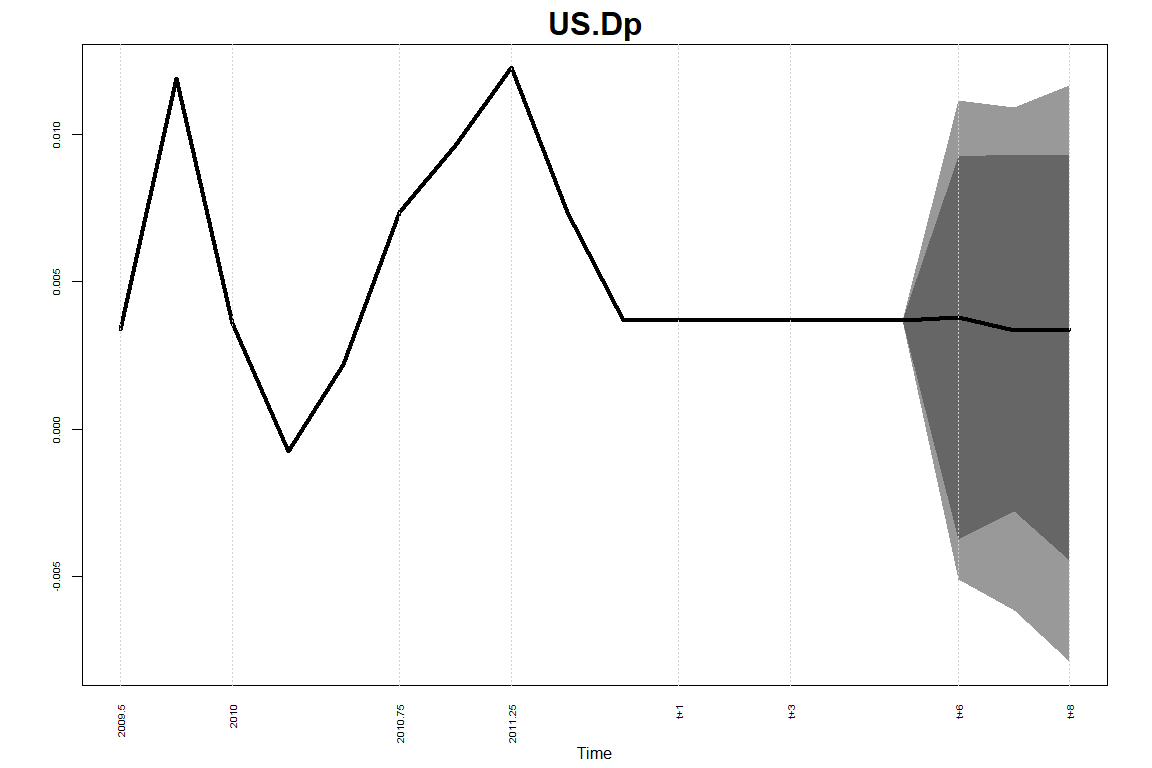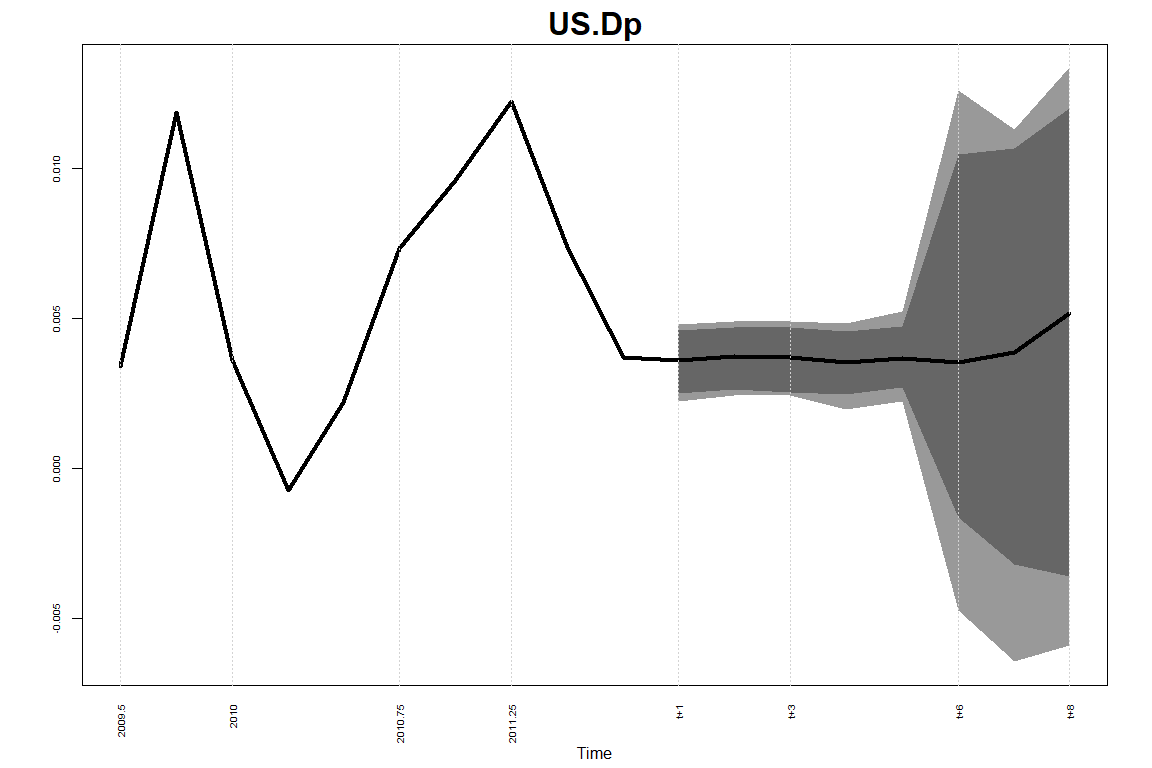Conditional forecast of US Inflation, top panel without uncertainty during the conditioning, bottom panel with uncertainty.

with Cut denoting the number of realized data points that should be shown in the plot prior the conditioning starts.

# Appendix

## Function Arguments bgvar

Main arguments and description of the function bgvar.

• Data: Either a

• list object of length $$N$$ that contains the data. Each element of the list refers to a country / entity. The number of columns (i.e., variables) in each country model can be different. The $$T$$ rows (i.e., number of time observations), however, need to be the same for each country. Country and variable names are not allowed to contain a . [dot].
• matrix of dimension $$T \times k$$, with $$k$$ denoting the sum of all endogenous variables of the system. The column names should consist of two parts, separated by a . The first part should denote the country / entity and the second part the name of the variable. Country and variable names are not allowed to contain a . [dot].
• W: An $$N \times N$$ weight matrix with 0 elements on the diagonal and row sums that sum up to unity or a list of weight matrices. See the help files for getweights for more details.

• plag: Number of lags used (the same for domestic, exogenous and weakly exogenous variables). Default set to plag=1.

• draws: Number of draws saved. Default set to draws=5000.

• burnin: Number of burn-ins. Default set to burnin=5000.

• prior: Either “SSVS”, “MN” or “NG”. See details below. Default set to prior=NG.

• SV: If set to "TRUE", models are fitted with stochastic volatility using the stochvol and GIGrvg packages. Due to storage issues, not the whole history of the $$T$$ variance covariance matrices are kept. Consequently, the BGVAR package shows only one set of impulse responses (with variance covariance matrix based on the median volatilities over the sample period) instead of $$T$$ sets. Specify SV=FALSE to turn SV off.

• hold.out: Defines the hold-out sample. Default without hold-out sample, thus set to zero.

• thin: Is a thinning interval which grabs every ’thin’th draw from the posterior output. For example, thin=10 saves every tenth draw from the posterior. Default set to thin=1.

• hyperpara: Is a list object that defines the hyperparameters when the prior is set to either "MN", "SSVS" or "NG".

• "miscellaneous:"
• a_1 is the prior hyperparameter for the inverted gamma prior (shape) (set a_1 = b_1 to a small value for the standard uninformative prior). Default is set to a_1=0.01.
• b_1 is the prior hyperparameter for the inverted gamma prior (rate). Default sit set to b_1=0.01.
• prmean is the prior mean on the first own lag of the autoregressive coefficient, standard value is prmean=1 for non-stationary data. The prior mean for the remaining autoregressive coefficients automatically set to 0.
• bmu If SV=TRUE, this is the prior hyperparameter for the mean of the log-volatilities. Default is bmu=0.
• Bmu If SV=TRUE, this is the prior hyperparameter for the variance of the mean of the log-volatilities. Default is Bmu=0.
• a0 If SV=TRUE, this is the hyperparameter for the Beta prior on the persistence parameter of the log-volatilities. Default is a0=25.
• b0 If SV=TRUE, this is the hyperparameter for the Beta prior on the persistence parameter of the log-volatilities. Default is b0=1.5.
• Bsigma If SV=TRUE, this is the hyperparameter for the Gamma prior on the variance of the log-volatilities. Default is Bsigma=1.
• "MN"
• shrink1 Starting value of shrink1. Default set to 0.1.
• shrink2 Starting value of shrink2. Default set to 0.2.
• shrink3 Hyperparameter of shrink3. Default set to 100.
• shrink4 Starting value of shrink4. Default set to 0.1.
• "SSVS"
• tau0 is the prior variance associated with the normal prior on the regression coefficients if a variable is NOT included (spike, tau0 should be close to zero).
• tau1 is the prior variance associated with the normal prior on the regression coefficients if a variable is included (slab, tau1 should be large).
• kappa0 is the prior variance associated with the normal prior on the covariances if a covariance equals zero (spike, kappa0 should be close to zero).
• kappa1 is the prior variance associated with the normal prior on the covariances if a covariance is unequal to zero (slab, kappa1 should be large).
• p_i is the prior inclusion probability for each regression coefficient (default is 0.5).
• q_ij is the prior inclusion probability for each covariance (default is 0.5).
• "NG"
• e_lambda Prior hyperparameter for the Gamma prior on the lag-specific shrinkage components, standard value is e_lambda=1.5.
• d_lambda Prior hyperparameter for the Gamma prior on the lag-specific shrinkage components, standard value is d_lambda=1.
• tau_theta Parameter of the Normal-Gamma prior that governs the heaviness of the tails of the prior distribution. A value of tau_theta=1 would lead to the Bayesian LASSO. Default value differs per entity and set to tau_theta=1/log(M), where M is the number of endogenous variables per entity.
• sample_tau If set to TRUE tau_theta is sampled.
• eigen Set to TRUE if you want to compute the largest eigenvalue of the companion matrix for each posterior draw. If the modulus of the eigenvalue is significantly larger than unity, the model is unstable. Unstable draws exceeding an eigenvalue of one are then excluded. If eigen is set to a numeric value, then this corresponds to the maximum eigenvalue. The default is set to $$1.05$$ (which excludes all posterior draws for which the eigenvalue of the companion matrix was larger than $$1.05$$ in modulus).

• Ex For including truly exogenous variables to the model. Either a + list object of maximum length N that contains the data. Each element of the list refers to a country/entity and has to match the country/entity names in Data. If no truly exogenous variables are added to the respective country/entity model, omit the entry. The T rows (i.e., number of time observations), however, need to be the same for each country. Country and variable names are not allowed to contain a . [dot] since this is our naming convention. + matrix object of dimension T times number of truly exogenous variables. The column names should consist of two parts, separated by a . [dot]. The first part should denote the country / entity name and the second part the name of the variable. Country and variable names are not allowed to contain a . [dot].

• trend If set to TRUE a deterministic trend is added to the country models.

• expert Expert settings, must be provided as list. Default is set to NULL. + variable.list In case W is a list of weight matrices, specify here which set of variables should be weighted by which weighting matrix. Default is set to NULL. + OE.weights: Default value is NULL. Can be used to provide information of how to handle additional country models (other entities). Additional country models can be used to endogenously determine variables that are (weakly) exogenous for the majority of the other country models. As examples, one could think of an additional oil price model (Mohaddes and Raissi 2019) or a model for the joint euro area monetary policy (Georgiadis 2015; Feldkircher, Gruber, and Huber 2020). The data for these additional country models has to be contained in Data. The number of additional country models is unlimited. Each list entry of OE.weights has to be named similar to the name of the additional country model contained in Data. Each slot of OE.weights has to contain the following information: + weights a vector of weights with names relating to the countries for which data should be aggregated. Can also relate to a subset of countries contained in the data. + variables a vector of variable names that should be included in the additional country model. Variables that are not contained in the data slot of the extra country model are assumed to be weakly exogenous for the additional country model (aggregated with weights). + exo a vector of variable names that should be fed into the other countries as (weakly) exogenous variables. + Wex.restr A character vector that contains variables that should only be specified as weakly exogenous if not contained as endogenous variable in a particular country. An example that has often been used in the literature is to place these restrictions on nominal exchange rates. Default is NULL in which case all weakly exogenous variables are treated symmetrically. See function getweights for more details. + save.country.store If set to TRUE then function also returns the container of all draws of the individual country models. Significantly raises object size of output and default is thus set to FALSE. + save.shrink.store If set to TRUE the function also inspects posterior output of shrinkage coefficients. Default set to FALSE. + save.vola.store If set to TRUE the function also inspects posterior output of coefficients associated with the volatility process. Default set to FALSE. + use_R Boolean whether estimation should fall back on R version, otherwise Rcpp version is used (default). + applyfun applyfun Allows for user-specific apply function, which has to have the same interface than . If cores=NULL then lapply is used, if set to a numeric either parallel::parLapply() is used on Windows platforms and parallel::mclapply() on non-Windows platforms. + cores Specifies the number of cores which should be used. Default is set to and is used.

• verbose If set to FALSE it suppresses printing messages to the console.

Below, find some example code for all three priors.

# load dataset
data(eerData)
# Minnesota prior and two different weight matrices and no SV
# weights for first variable set tradeW.0012, for second finW0711
variable.list      <- list()
variable.list$real <- c("y","Dp","tb") variable.list$fin  <- c("stir","ltir","rer")
Hyperparm.MN <- list(a_i = 0.01, # prior for the shape parameter of the IG
b_i = 0.01  # prior for the scale parameter of the IG
)
model.MN<-bgvar(Data=eerData,
draws=200,
burnin=200,
plag=1,
hyperpara=Hyperparm.MN,
prior="MN",
thin=1,
eigen=TRUE,
SV=TRUE,
expert=list(variable.list=variable.list))
# SSVS prior
Hyperparm.ssvs <- list(tau0   = 0.1,  # coefficients: prior variance for the spike
# (tau0 << tau1)
tau1   = 3,    # coefficients: prior variance for the slab
# (tau0 << tau1)
kappa0 = 0.1,  # covariances: prior variance for the spike
# (kappa0 << kappa1)
kappa1 = 7,    # covariances: prior variance for the slab
# (kappa0 << kappa1)
a_1    = 0.01, # prior for the shape parameter of the IG
b_1    = 0.01, # prior for the scale parameter of the IG
p_i    = 0.5,  # prior inclusion probability of coefficients
q_ij   = 0.5   # prior inclusion probability of covariances
)
model.ssvs<-bgvar(Data=eerData,
draws=100,
burnin=100,
plag=1,
hyperpara=Hyperparm.ssvs,
prior="SSVS",
thin=1,
eigen=TRUE)
# Normal Gamma prior
data(monthlyData)
monthlyData$OC<-NULL Hyperparm.ng<-list(d_lambda = 1.5, # coefficients: prior hyperparameter for the NG-prior e_lambda = 1, # coefficients: prior hyperparameter for the NG-prior prmean = 0, # prior mean for the first lag of the AR coefficients a_1 = 0.01, # prior for the shape parameter of the IG b_1 = 0.01, # prior for the scale parameter of the IG tau_theta = .6, # (hyper-)parameter for the NG sample_tau = FALSE # estimate a? ) model.ng<-bgvar(Data=monthlyData, W=W, draws=200, burnin=100, plag=1, hyperpara=Hyperparm.ng, prior="NG", thin=2, eigen=TRUE, SV=TRUE, expert=list(OE.weights=list(EB=EA.weights))) ## Function Arguments irf() • x: An objected fitted by function bgvar. • n.ahead: Forecasting horizon. • shockinfo Dataframe with additional information about the nature of shocks. Depending on the ident argument, the dataframe has to be specified differently. In order to get a dummy version for each identification scheme use get_shockinfo. • quantiles Numeric vector with posterior quantiles. Default is set to compute median along with 68%/80%/90% confidence intervals. • expert Expert settings, must be provided as list. Default is set to NULL. • MaxTries Numeric specifying maximal number of tries for finding a rotation matrix with sign-restrictions. Attention: setting this number very large may results in very long computation times. • save.store If set to TRUE the full posterior of both, impulse response and rotation matrices, are returned. Default is FALSE in order to save storage. • use_R Boolean whether IRF computation should fall back on R version, otherwise Rcpp version is used (default). • applyfun In case use_R=TRUE, this allows for user-specific apply function, which has to have the same interface as lapply. If cores=NULL then lapply is used, if set to a numeric either parallel::parLapply() is used on Windows platforms and parallel::mclapply() on non-Windows platforms. • cores Numeric specifying the number of cores which should be used, also all and half is possible. By default only one core is used. • verbose If set to FALSE it suppresses printing messages to the console. Below, find some further examples.  # First example, a US monetary policy shock, quarterly data library(BGVAR) data(eerData) model.eer<-bgvar(Data=eerData,W=W.trade0012,draws=500,burnin=500,plag=1,prior="SSVS",thin=10,eigen=TRUE,trend=TRUE) # generalized impulse responses shockinfo<-get_shockinfo("girf") shockinfo$shock<-"US.stir"; shockinfo$scale<--100 irf.girf.us.mp<-irf(model.eer, n.ahead=24, shockinfo=shockinfo) # cholesky identification shockinfo<-get_shockinfo("chol") shockinfo$shock<-"US.stir"; shockinfo\$scale<--100

# sign restrictions
shockinfo <- get_shockinfo("sign")
sign=c("<","<"), horizon=c(1,1), scale=1, prob=1)

# sign restrictions with relaxed cross-country restrictions
shockinfo <- get_shockinfo("sign")
# restriction for other countries holds to 75\%
sign=c("<","<","<"), horizon=1, scale=1, prob=c(1,0.75,0.75))
sign=c("<","<","<"), horizon=1, scale=1, prob=c(1,0.75,0.75))

# Example with zero restriction (Arias et al., 2018) and
# rationality conditions (D'Amico and King, 2017).
data("eerDataspf")
plag=1, prior="SSVS", eigen=TRUE)
shockinfo <- get_shockinfo("sign")
restriction=c("US.Dp_t+4","US.stir","US.y_t+4"),
sign=c("<","0","<"), horizon=1, prob=1, scale=1)
# rationality condition: US.stir_t+4 on impact is equal to average of
# IRF of US.stir between horizon 1 to 4
expert=list(MaxTries=10))
par(oldpar)